# Machine Learning Glossary

This glossary defines general machine learning terms, plus terms specific to TensorFlow.

## ablation

A technique for evaluating the importance of a feature or component by temporarily removing it from a model. You then retrain the model without that feature or component, and if the retrained model performs significantly worse, then the removed feature or component was likely important.

For example, suppose you train a classification model on 10 features and achieve 88% precision on the test set. To check the importance of the first feature, you can retrain the model using only the nine other features. If the retrained model performs significantly worse (for instance, 55% precision), then the removed feature was probably important. Conversely, if the retrained model performs equally well, then that feature was probably not that important.

Ablation can also help determine the importance of:

• Larger components, such as an entire subsystem of a larger ML system
• Processes or techniques, such as a data preprocessing step

In both cases, you would observe how the system's performance changes (or doesn't change) after you've removed the component.

## A/B testing

A statistical way of comparing two (or more) techniques—the A and the B. Typically, the A is an existing technique, and the B is a new technique. A/B testing not only determines which technique performs better but also whether the difference is statistically significant.

A/B testing usually compares a single metric on two techniques; for example, how does model accuracy compare for two techniques? However, A/B testing can also compare any finite number of metrics.

## accelerator chip

A category of specialized hardware components designed to perform key computations needed for deep learning algorithms.

Accelerator chips (or just accelerators, for short) can significantly increase the speed and efficiency of training and inference tasks compared to a general-purpose CPU. They are ideal for training neural networks and similar computationally intensive tasks.

Examples of accelerator chips include:

• Google's Tensor Processing Units (TPUs) with dedicated hardware for deep learning.
• NVIDIA's GPUs which, though initially designed for graphics processing, are designed to enable parallel processing, which can significantly increase processing speed.

## accuracy

#fundamentals

The number of correct classification predictions divided by the total number of predictions. That is:

$$\text{Accuracy} = \frac{\text{correct predictions}} {\text{correct predictions + incorrect predictions }}$$

For example, a model that made 40 correct predictions and 10 incorrect predictions would have an accuracy of:

$$\text{Accuracy} = \frac{\text{40}} {\text{40 + 10}} = \text{80%}$$

Binary classification provides specific names for the different categories of correct predictions and incorrect predictions. So, the accuracy formula for binary classification is as follows:

$$\text{Accuracy} = \frac{\text{TP} + \text{TN}} {\text{TP} + \text{TN} + \text{FP} + \text{FN}}$$

where:

Compare and contrast accuracy with precision and recall.

## action

#rl

In reinforcement learning, the mechanism by which the agent transitions between states of the environment. The agent chooses the action by using a policy.

## activation function

#fundamentals

A function that enables neural networks to learn nonlinear (complex) relationships between features and the label.

Popular activation functions include:

The plots of activation functions are never single straight lines. For example, the plot of the ReLU activation function consists of two straight lines:A plot of the sigmoid activation function looks as follows:## active learning

A training approach in which the algorithm chooses some of the data it learns from. Active learning is particularly valuable when labeled examples are scarce or expensive to obtain. Instead of blindly seeking a diverse range of labeled examples, an active learning algorithm selectively seeks the particular range of examples it needs for learning.

A sophisticated gradient descent algorithm that rescales the gradients of each parameter, effectively giving each parameter an independent learning rate. For a full explanation, see this paper.

## agent

#rl

In reinforcement learning, the entity that uses a policy to maximize the expected return gained from transitioning between states of the environment.

#clustering

## anomaly detection

The process of identifying outliers. For example, if the mean for a certain feature is 100 with a standard deviation of 10, then anomaly detection should flag a value of 200 as suspicious.

## AR

Abbreviation for augmented reality.

## artificial general intelligence

A non-human mechanism that demonstrates a broad range of problem solving, creativity, and adaptability. For example, a program demonstrating artificial general intelligence could translate text, compose symphonies, and excel at games that have not yet been invented.

## artificial intelligence

#fundamentals

A non-human program or model that can solve sophisticated tasks. For example, a program or model that translates text or a program or model that identifies diseases from radiologic images both exhibit artificial intelligence.

Formally, machine learning is a sub-field of artificial intelligence. However, in recent years, some organizations have begun using the terms artificial intelligence and machine learning interchangeably.

## attention

#language

A mechanism used in a neural network that indicates the importance of a particular word or part of a word. Attention compresses the amount of information a model needs to predict the next token/word. A typical attention mechanism might consist of a weighted sum over a set of inputs, where the weight for each input is computed by another part of the neural network.

Refer also to self-attention and multi-head self-attention, which are the building blocks of Transformers.

## attribute

#fairness

Synonym for feature.

In machine learning fairness, attributes often refer to characteristics pertaining to individuals.

## attribute sampling

#df

A tactic for training a decision forest in which each decision tree considers only a random subset of possible features when learning the condition. Generally, a different subset of features is sampled for each node. In contrast, when training a decision tree without attribute sampling, all possible features are considered for each node.

## AUC (Area under the ROC curve)

#fundamentals

A number between 0.0 and 1.0 representing a binary classification model's ability to separate positive classes from negative classes. The closer the AUC is to 1.0, the better the model's ability to separate classes from each other.

For example, the following illustration shows a classifier model that separates positive classes (green ovals) from negative classes (purple rectangles) perfectly. This unrealistically perfect model has an AUC of 1.0: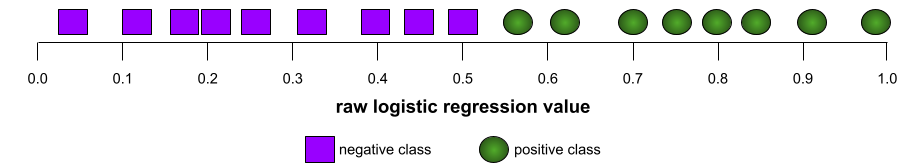Conversely, the following illustration shows the results for a classifier model that generated random results. This model has an AUC of 0.5: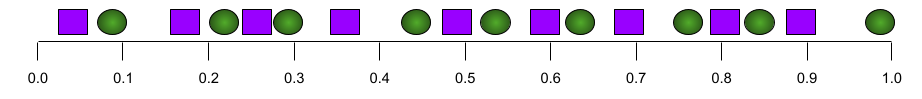Yes, the preceding model has an AUC of 0.5, not 0.0.

Most models are somewhere between the two extremes. For instance, the following model separates positives from negatives somewhat, and therefore has an AUC somewhere between 0.5 and 1.0: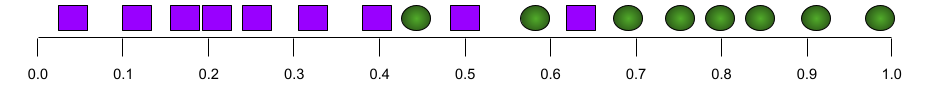AUC ignores any value you set for classification threshold. Instead, AUC considers all possible classification thresholds.

## augmented reality

#image

A technology that superimposes a computer-generated image on a user's view of the real world, thus providing a composite view.

## autoencoder

#language
#image

A system that learns to extract the most important information from the input. Autoencoders are a combination of an encoder and decoder. Autoencoders rely on the following two-step process:

1. The encoder maps the input to a (typically) lossy lower-dimensional (intermediate) format.
2. The decoder builds a lossy version of the original input by mapping the lower-dimensional format to the original higher-dimensional input format.

Autoencoders are trained end-to-end by having the decoder attempt to reconstruct the original input from the encoder's intermediate format as closely as possible. Because the intermediate format is smaller (lower-dimensional) than the original format, the autoencoder is forced to learn what information in the input is essential, and the output won't be perfectly identical to the input.

For example:

• If the input data is a graphic, the non-exact copy would be similar to the original graphic, but somewhat modified. For example, perhaps the non-exact copy removes noise from the original graphic or fills in some missing pixels.
• If the input data is text, an autoencoder would generate new text that mimics (but is not identical to) the original text.

## automation bias

#fairness

When a human decision maker favors recommendations made by an automated decision-making system over information made without automation, even when the automated decision-making system makes errors.

## AutoML

Any automated process for building machine learning models. AutoML can automatically do tasks such as the following:

AutoML is useful for data scientists because it can save them time and effort in developing machine learning pipelines and improve prediction accuracy. It is also useful to non-experts, by making complicated machine learning tasks more accessible to them.

## auto-regressive model

#language
#image
#generativeAI

A model that infers a prediction based on its own previous predictions. For example, auto-regressive language models predict the next token based on the previously predicted tokens. All Transformer-based large language models are auto-regressive.

In contrast, GAN-based image models are usually not auto-regressive since they generate an image in a single forward-pass and not iteratively in steps. However, certain image generation models are auto-regressive because they generate an image in steps.

## auxiliary loss

A loss function—used in conjunction with a neural network model’s main loss function—that helps accelerate training during the early iterations when weights are randomly initialized.

Auxiliary loss functions push effective gradients to the earlier layers. This facilitates convergence during training by combating the vanishing gradient problem.

## average precision

A metric for summarizing the performance of a ranked sequence of results. Average precision is calculated by taking the average of the precision values for each relevant result (each result in the ranked list where the recall increases relative to the previous result).

## axis-aligned condition

#df

In a decision tree, a condition that involves only a single feature. For example, if area is a feature, then the following is an axis-aligned condition:

area > 200


Contrast with oblique condition.

## backpropagation

#fundamentals

The algorithm that implements gradient descent in neural networks.

Training a neural network involves many iterations of the following two-pass cycle:

1. During the forward pass, the system processes a batch of examples to yield prediction(s). The system compares each prediction to each label value. The difference between the prediction and the label value is the loss for that example. The system aggregates the losses for all the examples to compute the total loss for the current batch.
2. During the backward pass (backpropagation), the system reduces loss by adjusting the weights of all the neurons in all the hidden layer(s).

Neural networks often contain many neurons across many hidden layers. Each of those neurons contribute to the overall loss in different ways. Backpropagation determines whether to increase or decrease the weights applied to particular neurons.

The learning rate is a multiplier that controls the degree to which each backward pass increases or decreases each weight. A large learning rate will increase or decrease each weight more than a small learning rate.

In calculus terms, backpropagation implements calculus' chain rule. That is, backpropagation calculates the partial derivative of the error with respect to each parameter. For more details, see this tutorial in Machine Learning Crash Course.

Years ago, ML practitioners had to write code to implement backpropagation. Modern ML APIs like TensorFlow now implement backpropagation for you. Phew!

## bagging

#df

A method to train an ensemble where each constituent model trains on a random subset of training examples sampled with replacement. For example, a random forest is a collection of decision trees trained with bagging.

The term bagging is short for bootstrap aggregating.

## bag of words

#language

A representation of the words in a phrase or passage, irrespective of order. For example, bag of words represents the following three phrases identically:

• the dog jumps
• jumps the dog
• dog jumps the

Each word is mapped to an index in a sparse vector, where the vector has an index for every word in the vocabulary. For example, the phrase the dog jumps is mapped into a feature vector with non-zero values at the three indices corresponding to the words the, dog, and jumps. The non-zero value can be any of the following:

• A 1 to indicate the presence of a word.
• A count of the number of times a word appears in the bag. For example, if the phrase were the maroon dog is a dog with maroon fur, then both maroon and dog would be represented as 2, while the other words would be represented as 1.
• Some other value, such as the logarithm of the count of the number of times a word appears in the bag.

## baseline

A model used as a reference point for comparing how well another model (typically, a more complex one) is performing. For example, a logistic regression model might serve as a good baseline for a deep model.

For a particular problem, the baseline helps model developers quantify the minimal expected performance that a new model must achieve for the new model to be useful.

## batch

#fundamentals

The set of examples used in one training iteration. The batch size determines the number of examples in a batch.

See epoch for an explanation of how a batch relates to an epoch.

## batch inference

#TensorFlow

The process of inferring predictions on multiple unlabeled examples divided into smaller subsets ("batches").

Batch inference can leverage the parallelization features of accelerator chips. That is, multiple accelerators can simultaneously infer predictions on different batches of unlabeled examples, dramatically increasing the number of inferences per second.

## batch normalization

Normalizing the input or output of the activation functions in a hidden layer. Batch normalization can provide the following benefits:

## batch size

#fundamentals

The number of examples in a batch. For instance, if the batch size is 100, then the model processes 100 examples per iteration.

The following are popular batch size strategies:

• Stochastic Gradient Descent (SGD), in which the batch size is 1.
• full batch, in which the batch size is the number of examples in the entire training set. For instance, if the training set contains a million examples, then the batch size would be a million examples. Full batch is usually an inefficient strategy.
• mini-batch in which the batch size is usually between 10 and 1000. Mini-batch is usually the most efficient strategy.

## Bayesian neural network

A probabilistic neural network that accounts for uncertainty in weights and outputs. A standard neural network regression model typically predicts a scalar value; for example, a standard model predicts a house price of 853,000. In contrast, a Bayesian neural network predicts a distribution of values; for example, a Bayesian model predicts a house price of 853,000 with a standard deviation of 67,200.

A Bayesian neural network relies on Bayes' Theorem to calculate uncertainties in weights and predictions. A Bayesian neural network can be useful when it is important to quantify uncertainty, such as in models related to pharmaceuticals. Bayesian neural networks can also help prevent overfitting.

## Bayesian optimization

A probabilistic regression model technique for optimizing computationally expensive objective functions by instead optimizing a surrogate that quantifies the uncertainty via a Bayesian learning technique. Since Bayesian optimization is itself very expensive, it is usually used to optimize expensive-to-evaluate tasks that have a small number of parameters, such as selecting hyperparameters.

## Bellman equation

#rl

In reinforcement learning, the following identity satisfied by the optimal Q-function:

$Q(s, a) = r(s, a) + \gamma \mathbb{E}_{s'|s,a} \max_{a'} Q(s', a')$

Reinforcement learning algorithms apply this identity to create Q-learning via the following update rule:

$Q(s,a) \gets Q(s,a) + \alpha \left[r(s,a) + \gamma \displaystyle\max_{\substack{a_1}} Q(s’,a’) - Q(s,a) \right]$

Beyond reinforcement learning, the Bellman equation has applications to dynamic programming. See the Wikipedia entry for Bellman Equation.

## BERT (Bidirectional Encoder Representations from Transformers)

#language

A model architecture for text representation. A trained BERT model can act as part of a larger model for text classification or other ML tasks.

BERT has the following characteristics:

BERT's variants include:

See Open Sourcing BERT: State-of-the-Art Pre-training for Natural Language Processing for an overview of BERT.

## bias (ethics/fairness)

#fairness
#fundamentals

1. Stereotyping, prejudice or favoritism towards some things, people, or groups over others. These biases can affect collection and interpretation of data, the design of a system, and how users interact with a system. Forms of this type of bias include:

2. Systematic error introduced by a sampling or reporting procedure. Forms of this type of bias include:

Not to be confused with the bias term in machine learning models or prediction bias.

## bias (math) or bias term

#fundamentals

An intercept or offset from an origin. Bias is a parameter in machine learning models, which is symbolized by either of the following:

• b
• w0

For example, bias is the b in the following formula:

$$y' = b + w_1x_1 + w_2x_2 + … w_nx_n$$

In a simple two-dimensional line, bias just means "y-intercept." For example, the bias of the line in the following illustration is 2.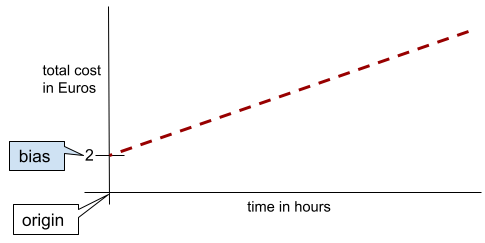Bias exists because not all models start from the origin (0,0). For example, suppose an amusement park costs 2 Euros to enter and an additional 0.5 Euro for every hour a customer stays. Therefore, a model mapping the total cost has a bias of 2 because the lowest cost is 2 Euros.

Bias is not to be confused with bias in ethics and fairness or prediction bias.

## bidirectional

#language

A term used to describe a system that evaluates the text that both precedes and follows a target section of text. In contrast, a unidirectional system only evaluates the text that precedes a target section of text.

For example, consider a masked language model that must determine probabilities for the word or words representing the underline in the following question:

What is the _____ with you?

A unidirectional language model would have to base its probabilities only on the context provided by the words "What", "is", and "the". In contrast, a bidirectional language model could also gain context from "with" and "you", which might help the model generate better predictions.

## bidirectional language model

#language

A language model that determines the probability that a given token is present at a given location in an excerpt of text based on the preceding and following text.

## bigram

#seq
#language

An N-gram in which N=2.

## binary classification

#fundamentals

A type of classification task that predicts one of two mutually exclusive classes:

For example, the following two machine learning models each perform binary classification:

• A model that determines whether email messages are spam (the positive class) or not spam (the negative class).
• A model that evaluates medical symptoms to determine whether a person has a particular disease (the positive class) or doesn't have that disease (the negative class).

Contrast with multi-class classification.

## binary condition

#df

In a decision tree, a condition that has only two possible outcomes, typically yes or no. For example, the following is a binary condition:

temperature >= 100


Contrast with non-binary condition.

## binning

Synonym for bucketing.

## BLEU (Bilingual Evaluation Understudy)

#language

A score between 0.0 and 1.0, inclusive, indicating the quality of a translation between two human languages (for example, between English and Russian). A BLEU score of 1.0 indicates a perfect translation; a BLEU score of 0.0 indicates a terrible translation.

## boosting

A machine learning technique that iteratively combines a set of simple and not very accurate classifiers (referred to as "weak" classifiers) into a classifier with high accuracy (a "strong" classifier) by upweighting the examples that the model is currently misclassifying.

## bounding box

#image

In an image, the (x, y) coordinates of a rectangle around an area of interest, such as the dog in the image below.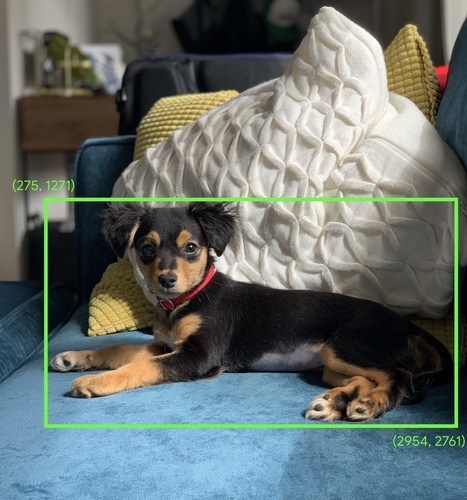Expanding the shape of an operand in a matrix math operation to dimensions compatible for that operation. For instance, linear algebra requires that the two operands in a matrix addition operation must have the same dimensions. Consequently, you can't add a matrix of shape (m, n) to a vector of length n. Broadcasting enables this operation by virtually expanding the vector of length n to a matrix of shape (m, n) by replicating the same values down each column.

For example, given the following definitions, linear algebra prohibits A+B because A and B have different dimensions:

A = [[7, 10, 4],
[13, 5, 9]]
B = 


However, broadcasting enables the operation A+B by virtually expanding B to:

 [[2, 2, 2],
[2, 2, 2]]


Thus, A+B is now a valid operation:

[[7, 10, 4],  +  [[2, 2, 2],  =  [[ 9, 12, 6],
[13, 5, 9]]      [2, 2, 2]]      [15, 7, 11]]


See the following description of broadcasting in NumPy for more details.

## bucketing

#fundamentals

Converting a single feature into multiple binary features called buckets or bins, typically based on a value range. The chopped feature is typically a continuous feature.

For example, instead of representing temperature as a single continuous floating-point feature, you could chop ranges of temperatures into discrete buckets, such as:

• <= 10 degrees Celsius would be the "cold" bucket.
• 11 - 24 degrees Celsius would be the "temperate" bucket.
• >= 25 degrees Celsius would be the "warm" bucket.

The model will treat every value in the same bucket identically. For example, the values 13 and 22 are both in the temperate bucket, so the model treats the two values identically.

## calibration layer

A post-prediction adjustment, typically to account for prediction bias. The adjusted predictions and probabilities should match the distribution of an observed set of labels.

## candidate generation

#recsystems

The initial set of recommendations chosen by a recommendation system. For example, consider a bookstore that offers 100,000 titles. The candidate generation phase creates a much smaller list of suitable books for a particular user, say 500. But even 500 books is way too many to recommend to a user. Subsequent, more expensive, phases of a recommendation system (such as scoring and re-ranking) reduce those 500 to a much smaller, more useful set of recommendations.

## candidate sampling

A training-time optimization that calculates a probability for all the positive labels, using, for example, softmax, but only for a random sample of negative labels. For instance, given an example labeled beagle and dog, candidate sampling computes the predicted probabilities and corresponding loss terms for:

• beagle
• dog
• a random subset of the remaining negative classes (for example, cat, lollipop, fence).

The idea is that the negative classes can learn from less frequent negative reinforcement as long as positive classes always get proper positive reinforcement, and this is indeed observed empirically.

Candidate sampling is more computationally efficient than training algorithms that compute predictions for all negative classes, particularly when the number of negative classes is very large.

## categorical data

#fundamentals

Features having a specific set of possible values. For example, consider a categorical feature named traffic-light-state, which can only have one of the following three possible values:

• red
• yellow
• green

By representing traffic-light-state as a categorical feature, a model can learn the differing impacts of red, green, and yellow on driver behavior.

Categorical features are sometimes called discrete features.

Contrast with numerical data.

## causal language model

#language

Synonym for unidirectional language model.

See bidirectional language model to contrast different directional approaches in language modeling.

## centroid

#clustering

The center of a cluster as determined by a k-means or k-median algorithm. For instance, if k is 3, then the k-means or k-median algorithm finds 3 centroids.

## centroid-based clustering

#clustering

A category of clustering algorithms that organizes data into nonhierarchical clusters. k-means is the most widely used centroid-based clustering algorithm.

Contrast with hierarchical clustering algorithms.

## chain-of-thought prompting

#language
#generativeAI

A prompt engineering technique that encourages a large language model (LLM) to explain its reasoning, step by step. For example, consider the following prompt, paying particular attention to the second sentence:

How many g forces would a driver experience in a car that goes from 0 to 60 miles per hour in 7 seconds? In the answer, show all relevant calculations.

The LLM's response would likely:

• Show a sequence of physics formulas, plugging in the values 0, 60, and 7 in appropriate places.
• Explain why it chose those formulas and what the various variables mean.

Chain-of-thought prompting forces the LLM to perform all the calculations, which might lead to a more correct answer. In addition, chain-of-thought prompting enables the user to examine the LLM's steps to determine whether or not the answer makes sense.

## checkpoint

Data that captures the state of a model's parameters at a particular training iteration. Checkpoints enable exporting model weights, or performing training across multiple sessions. Checkpoints also enable training to continue past errors (for example, job preemption).

When fine tuning, the starting point for training the new model will be a specific checkpoint of the pre-trained model.

## class

#fundamentals

A category that a label can belong to. For example:

A classification model predicts a class. In contrast, a regression model predicts a number rather than a class.

## classification model

#fundamentals

A model whose prediction is a class. For example, the following are all classification models:

• A model that predicts an input sentence's language (French? Spanish? Italian?).
• A model that predicts tree species (Maple? Oak? Baobab?).
• A model that predicts the positive or negative class for a particular medical condition.

In contrast, regression models predict numbers rather than classes.

Two common types of classification models are:

## classification threshold

#fundamentals

In a binary classification, a number between 0 and 1 that converts the raw output of a logistic regression model into a prediction of either the positive class or the negative class. Note that the classification threshold is a value that a human chooses, not a value chosen by model training.

A logistic regression model outputs a raw value between 0 and 1. Then:

• If this raw value is greater than the classification threshold, then the positive class is predicted.
• If this raw value is less than the classification threshold, then the negative class is predicted.

For example, suppose the classification threshold is 0.8. If the raw value is 0.9, then the model predicts the positive class. If the raw value is 0.7, then the model predicts the negative class.

The choice of classification threshold strongly influences the number of false positives and false negatives.

## class-imbalanced dataset

#fundamentals

A dataset for a classification problem in which the total number of labels of each class differs significantly. For example, consider a binary classification dataset whose two labels are divided as follows:

• 1,000,000 negative labels
• 10 positive labels

The ratio of negative to positive labels is 100,000 to 1, so this is a class-imbalanced dataset.

In contrast, the following dataset is not class-imbalanced because the ratio of negative labels to positive labels is relatively close to 1:

• 517 negative labels
• 483 positive labels

Multi-class datasets can also be class-imbalanced. For example, the following multi-class classification dataset is also class-imbalanced because one label has far more examples than the other two:

• 1,000,000 labels with class "green"
• 200 labels with class "purple"
• 350 labels with class "orange"

## clipping

#fundamentals

A technique for handling outliers by doing either or both of the following:

• Reducing feature values that are greater than a maximum threshold down to that maximum threshold.
• Increasing feature values that are less than a minimum threshold up to that minimum threshold.

For example, suppose that <0.5% of values for a particular feature fall outside the range 40–60. In this case, you could do the following:

• Clip all values over 60 (the maximum threshold) to be exactly 60.
• Clip all values under 40 (the minimum threshold) to be exactly 40.

Outliers can damage models, sometimes causing weights to overflow during training. Some outliers can also dramatically spoil metrics like accuracy. Clipping is a common technique to limit the damage.

## Cloud TPU

#TensorFlow

A specialized hardware accelerator designed to speed up machine learning workloads on Google Cloud Platform.

## clustering

#clustering

Grouping related examples, particularly during unsupervised learning. Once all the examples are grouped, a human can optionally supply meaning to each cluster.

Many clustering algorithms exist. For example, the k-means algorithm clusters examples based on their proximity to a centroid, as in the following diagram:A human researcher could then review the clusters and, for example, label cluster 1 as "dwarf trees" and cluster 2 as "full-size trees."

As another example, consider a clustering algorithm based on an example's distance from a center point, illustrated as follows:When neurons predict patterns in training data by relying almost exclusively on outputs of specific other neurons instead of relying on the network's behavior as a whole. When the patterns that cause co-adaption are not present in validation data, then co-adaptation causes overfitting. Dropout regularization reduces co-adaptation because dropout ensures neurons cannot rely solely on specific other neurons.

## collaborative filtering

#recsystems

Making predictions about the interests of one user based on the interests of many other users. Collaborative filtering is often used in recommendation systems.

## condition

#df

In a decision tree, any node that evaluates an expression. For example, the following portion of a decision tree contains two conditions: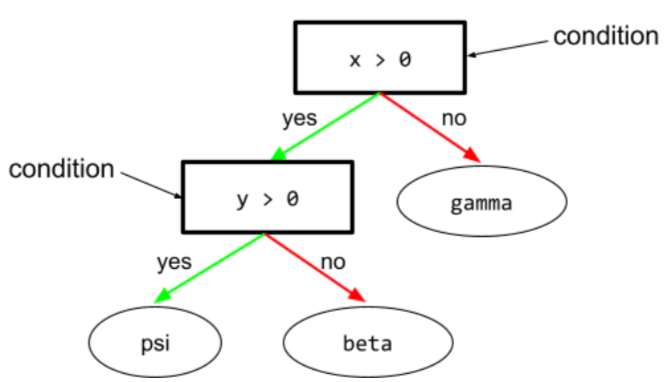A condition is also called a split or a test.

Contrast condition with leaf.

## configuration

The process of assigning the initial property values used to train a model, including:

In machine learning projects, configuration can be done through a special configuration file or via configuration libraries such as the following:

## confirmation bias

#fairness

The tendency to search for, interpret, favor, and recall information in a way that confirms one's preexisting beliefs or hypotheses. Machine learning developers may inadvertently collect or label data in ways that influence an outcome supporting their existing beliefs. Confirmation bias is a form of implicit bias.

Experimenter's bias is a form of confirmation bias in which an experimenter continues training models until a preexisting hypothesis is confirmed.

## confusion matrix

#fundamentals

An NxN table that summarizes the number of correct and incorrect predictions that a classification model made. For example, consider the following confusion matrix for a binary classification model:

Tumor (predicted) Non-Tumor (predicted)
Tumor (ground truth) 18 (TP) 1 (FN)
Non-Tumor (ground truth) 6 (FP) 452 (TN)

The preceding confusion matrix shows the following:

• Of the 19 predictions in which ground truth was Tumor, the model correctly classified 18 and incorrectly classified 1.
• Of the 458 predictions in which ground truth was Non-Tumor, the model correctly classified 452 and incorrectly classified 6.

The confusion matrix for a multi-class classification problem can help you identify patterns of mistakes. For example, consider the following confusion matrix for a 3-class multi-class classification model that categorizes three different iris types (Virginica, Versicolor, and Setosa). When the ground truth was Virginica, the confusion matrix shows that the model was far more likely to mistakenly predict Versicolor than Setosa:

Setosa (predicted) Versicolor (predicted) Virginica (predicted)
Setosa (ground truth) 88 12 0
Versicolor (ground truth) 6 141 7
Virginica (ground truth) 2 27 109

As yet another example, a confusion matrix could reveal that a model trained to recognize handwritten digits tends to mistakenly predict 9 instead of 4, or mistakenly predict 1 instead of 7.

Confusion matrices contain sufficient information to calculate a variety of performance metrics, including precision and recall.

## constituency parsing

#language

Dividing a sentence into smaller grammatical structures ("constituents"). A later part of the ML system, such as a natural language understanding model, can parse the constituents more easily than the original sentence. For example, consider the following sentence:

A constituency parser can divide this sentence into the following two constituents:

• My friend is a noun phrase.
• adopted two cats is a verb phrase.

These constituents can be further subdivided into smaller constituents. For example, the verb phrase

could be further subdivided into:

• two cats is another noun phrase.

## continuous feature

#fundamentals

A floating-point feature with an infinite range of possible values, such as temperature or weight.

Contrast with discrete feature.

## convenience sampling

Using a dataset not gathered scientifically in order to run quick experiments. Later on, it's essential to switch to a scientifically gathered dataset.

## convergence

#fundamentals

A state reached when loss values change very little or not at all with each iteration. For example, the following loss curve suggests convergence at around 700 iterations: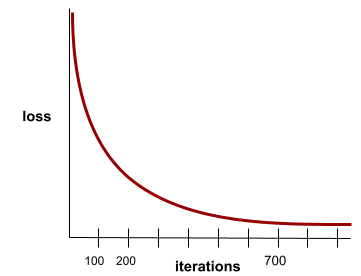A model converges when additional training won't improve the model.

In deep learning, loss values sometimes stay constant or nearly so for many iterations before finally descending. During a long period of constant loss values, you may temporarily get a false sense of convergence.

## convex function

A function in which the region above the graph of the function is a convex set. The prototypical convex function is shaped something like the letter U. For example, the following are all convex functions: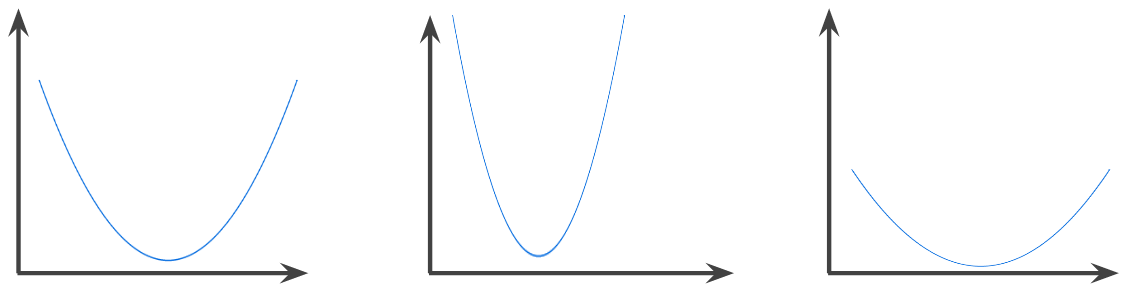In contrast, the following function is not convex. Notice how the region above the graph is not a convex set:A strictly convex function has exactly one local minimum point, which is also the global minimum point. The classic U-shaped functions are strictly convex functions. However, some convex functions (for example, straight lines) are not U-shaped.

## convex optimization

The process of using mathematical techniques such as gradient descent to find the minimum of a convex function. A great deal of research in machine learning has focused on formulating various problems as convex optimization problems and in solving those problems more efficiently.

For complete details, see Boyd and Vandenberghe, Convex Optimization.

## convex set

A subset of Euclidean space such that a line drawn between any two points in the subset remains completely within the subset. For instance, the following two shapes are convex sets:In contrast, the following two shapes are not convex sets:## convolution

#image

In mathematics, casually speaking, a mixture of two functions. In machine learning, a convolution mixes the convolutional filter and the input matrix in order to train weights.

The term "convolution" in machine learning is often a shorthand way of referring to either convolutional operation or convolutional layer.

Without convolutions, a machine learning algorithm would have to learn a separate weight for every cell in a large tensor. For example, a machine learning algorithm training on 2K x 2K images would be forced to find 4M separate weights. Thanks to convolutions, a machine learning algorithm only has to find weights for every cell in the convolutional filter, dramatically reducing the memory needed to train the model. When the convolutional filter is applied, it is simply replicated across cells such that each is multiplied by the filter.

## convolutional filter

#image

One of the two actors in a convolutional operation. (The other actor is a slice of an input matrix.) A convolutional filter is a matrix having the same rank as the input matrix, but a smaller shape. For example, given a 28x28 input matrix, the filter could be any 2D matrix smaller than 28x28.

In photographic manipulation, all the cells in a convolutional filter are typically set to a constant pattern of ones and zeroes. In machine learning, convolutional filters are typically seeded with random numbers and then the network trains the ideal values.

## convolutional layer

#image

A layer of a deep neural network in which a convolutional filter passes along an input matrix. For example, consider the following 3x3 convolutional filter:The following animation shows a convolutional layer consisting of 9 convolutional operations involving the 5x5 input matrix. Notice that each convolutional operation works on a different 3x3 slice of the input matrix. The resulting 3x3 matrix (on the right) consists of the results of the 9 convolutional operations: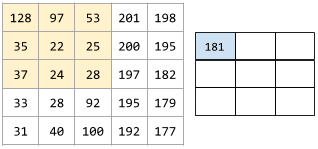## convolutional neural network

#image

A neural network in which at least one layer is a convolutional layer. A typical convolutional neural network consists of some combination of the following layers:

Convolutional neural networks have had great success in certain kinds of problems, such as image recognition.

## convolutional operation

#image

The following two-step mathematical operation:

1. Element-wise multiplication of the convolutional filter and a slice of an input matrix. (The slice of the input matrix has the same rank and size as the convolutional filter.)
2. Summation of all the values in the resulting product matrix.

For example, consider the following 5x5 input matrix:Now imagine the following 2x2 convolutional filter:Each convolutional operation involves a single 2x2 slice of the input matrix. For instance, suppose we use the 2x2 slice at the top-left of the input matrix. So, the convolution operation on this slice looks as follows:A convolutional layer consists of a series of convolutional operations, each acting on a different slice of the input matrix.

## cost

Synonym for loss.

## co-training

A semi-supervised learning approach particularly useful when all of the following conditions are true:

Co-training essentially amplifies independent signals into a stronger signal. For instance, consider a classification model that categorizes individual used cars as either Good or Bad. One set of predictive features might focus on aggregate characteristics such as the year, make, and model of the car; another set of predictive features might focus on the previous owner's driving record and the car's maintenance history.

The seminal paper on co-training is Combining Labeled and Unlabeled Data with Co-Training by Blum and Mitchell.

## counterfactual fairness

#fairness
A fairness metric that checks whether a classifier produces the same result for one individual as it does for another individual who is identical to the first, except with respect to one or more sensitive attributes. Evaluating a classifier for counterfactual fairness is one method for surfacing potential sources of bias in a model.

See "When Worlds Collide: Integrating Different Counterfactual Assumptions in Fairness" for a more detailed discussion of counterfactual fairness.

## coverage bias

#fairness

See selection bias.

## crash blossom

#language

A sentence or phrase with an ambiguous meaning. Crash blossoms present a significant problem in natural language understanding. For example, the headline Red Tape Holds Up Skyscraper is a crash blossom because an NLU model could interpret the headline literally or figuratively.

## critic

#rl

Synonym for Deep Q-Network.

## cross-entropy

A generalization of Log Loss to multi-class classification problems. Cross-entropy quantifies the difference between two probability distributions. See also perplexity.

## cross-validation

A mechanism for estimating how well a model would generalize to new data by testing the model against one or more non-overlapping data subsets withheld from the training set.

## data analysis

Obtaining an understanding of data by considering samples, measurement, and visualization. Data analysis can be particularly useful when a dataset is first received, before one builds the first model. It is also crucial in understanding experiments and debugging problems with the system.

## data augmentation

#image

Artificially boosting the range and number of training examples by transforming existing examples to create additional examples. For example, suppose images are one of your features, but your dataset doesn't contain enough image examples for the model to learn useful associations. Ideally, you'd add enough labeled images to your dataset to enable your model to train properly. If that's not possible, data augmentation can rotate, stretch, and reflect each image to produce many variants of the original picture, possibly yielding enough labeled data to enable excellent training.

## DataFrame

#fundamentals

A popular pandas datatype for representing datasets in memory.

A DataFrame is analogous to a table or a spreadsheet. Each column of a DataFrame has a name (a header), and each row is identified by a unique number.

Each column in a DataFrame is structured like a 2D array, except that each column can be assigned its own data type.

## data parallelism

A way of scaling training or inference that replicates an entire model onto multiple devices and then passes a subset of the input data to each device. Data parallelism can enable training and inference on very large batch sizes; however, data parallelism requires that the model be small enough to fit on all devices.

Data parallelism typically speeds training and inference.

## data set or dataset

#fundamentals

A collection of raw data, commonly (but not exclusively) organized in one of the following formats:

• a file in CSV (comma-separated values) format

## Dataset API (tf.data)

#TensorFlow

A high-level TensorFlow API for reading data and transforming it into a form that a machine learning algorithm requires. A tf.data.Dataset object represents a sequence of elements, in which each element contains one or more Tensors. A tf.data.Iterator object provides access to the elements of a Dataset.

For details about the Dataset API, see tf.data: Build TensorFlow input pipelines in the TensorFlow Programmer's Guide.

## decision boundary

The separator between classes learned by a model in a binary class or multi-class classification problems. For example, in the following image representing a binary classification problem, the decision boundary is the frontier between the orange class and the blue class:## decision forest

#df

A model created from multiple decision trees. A decision forest makes a prediction by aggregating the predictions of its decision trees. Popular types of decision forests include random forests and gradient boosted trees.

## decision threshold

Synonym for classification threshold.

## decision tree

#df

A supervised learning model composed of a set of conditions and leaves organized hierarchically. For example, the following is a decision tree: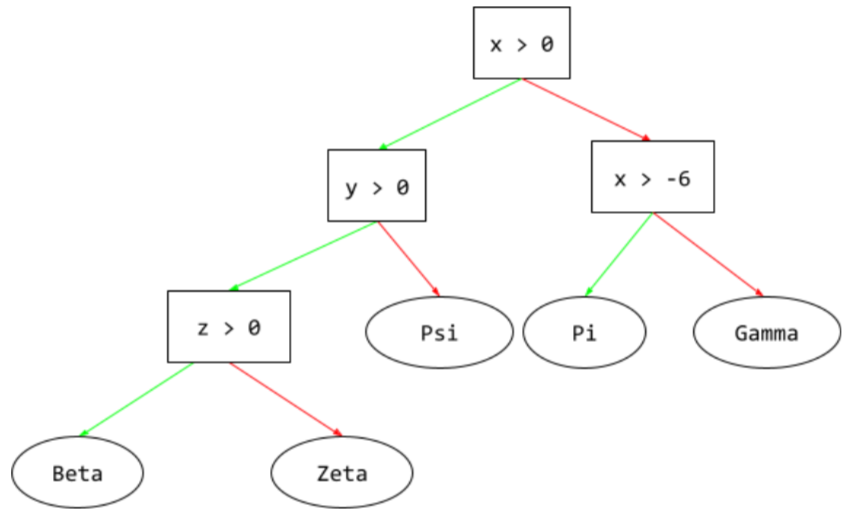## decoder

#language

In general, any ML system that converts from a processed, dense, or internal representation to a more raw, sparse, or external representation.

Decoders are often a component of a larger model, where they are frequently paired with an encoder.

In sequence-to-sequence tasks, a decoder starts with the internal state generated by the encoder to predict the next sequence.

Refer to Transformer for the definition of a decoder within the Transformer architecture.

## deep model

#fundamentals

A neural network containing more than one hidden layer.

A deep model is also called a deep neural network.

Contrast with wide model.

## deep neural network

Synonym for deep model.

## Deep Q-Network (DQN)

#rl

In Q-learning, a deep neural network that predicts Q-functions.

Critic is a synonym for Deep Q-Network.

## demographic parity

#fairness

A fairness metric that is satisfied if the results of a model's classification are not dependent on a given sensitive attribute.

For example, if both Lilliputians and Brobdingnagians apply to Glubbdubdrib University, demographic parity is achieved if the percentage of Lilliputians admitted is the same as the percentage of Brobdingnagians admitted, irrespective of whether one group is on average more qualified than the other.

Contrast with equalized odds and equality of opportunity, which permit classification results in aggregate to depend on sensitive attributes, but do not permit classification results for certain specified ground-truth labels to depend on sensitive attributes. See "Attacking discrimination with smarter machine learning" for a visualization exploring the tradeoffs when optimizing for demographic parity.

## denoising

#language

A common approach to self-supervised learning in which:

1. Noise is artificially added to the dataset.
2. The model tries to remove the noise.

Denoising enables learning from unlabeled examples. The original dataset serves as the target or label and the noisy data as the input.

Some masked language models use denoising as follows:

1. Noise is artificially added to an unlabeled sentence by masking some of the tokens.
2. The model tries to predict the original tokens.

## dense feature

#fundamentals

A feature in which most or all values are nonzero, typically a Tensor of floating-point values. For example, the following 10-element Tensor is dense because 9 of its values are nonzero:

 8 3 7 5 2 4 0 4 9 6

Contrast with sparse feature.

## dense layer

Synonym for fully connected layer.

## depth

#fundamentals

The sum of the following in a neural network:

For example, a neural network with five hidden layers and one output layer has a depth of 6.

Notice that the input layer doesn't influence depth.

## depthwise separable convolutional neural network (sepCNN)

#image

A convolutional neural network architecture based on Inception, but where Inception modules are replaced with depthwise separable convolutions. Also known as Xception.

A depthwise separable convolution (also abbreviated as separable convolution) factors a standard 3-D convolution into two separate convolution operations that are more computationally efficient: first, a depthwise convolution, with a depth of 1 (n ✕ n ✕ 1), and then second, a pointwise convolution, with length and width of 1 (1 ✕ 1 ✕ n).

## derived label

Synonym for proxy label.

## device

#TensorFlow

An overloaded term with the following two possible definitions:

1. A category of hardware that can run a TensorFlow session, including CPUs, GPUs, and TPUs.
2. When training an ML model on accelerator chips (GPUs or TPUs), the part of the system that actually manipulates tensors and embeddings. The device runs on accelerator chips. In contrast, the host typically runs on a CPU.

## differential privacy

An anonymization approach to privacy that protects an individual's personal information that might be included in a model’s training set. This approach ensures that the model doesn't infer much about a specific individual. Differential privacy injects noise during training to obscure individual data points.

Differential privacy is also used outside of machine learning. For example, data scientists sometimes use differential privacy to protect individual privacy when computing product usage statistics for different demographics.

## dimension reduction

Decreasing the number of dimensions used to represent a particular feature in a feature vector, typically by converting to an embedding vector.

## dimensions

Overloaded term having any of the following definitions:

• The number of levels of coordinates in a Tensor. For example:

• A scalar has zero dimensions; for example, ["Hello"].
• A vector has one dimension; for example, [3, 5, 7, 11].
• A matrix has two dimensions; for example, [[2, 4, 18], [5, 7, 14]].

You can uniquely specify a particular cell in a one-dimensional vector with one coordinate; you need two coordinates to uniquely specify a particular cell in a two-dimensional matrix.

• The number of entries in a feature vector.

• The number of elements in an embedding layer.

## direct prompting

#language
#generativeAI

Synonym for zero-shot prompting.

## discrete feature

#fundamentals

A feature with a finite set of possible values. For example, a feature whose values may only be animal, vegetable, or mineral is a discrete (or categorical) feature.

Contrast with continuous feature.

## discriminative model

A model that predicts labels from a set of one or more features. More formally, discriminative models define the conditional probability of an output given the features and weights; that is:

p(output | features, weights)


For example, a model that predicts whether an email is spam from features and weights is a discriminative model.

The vast majority of supervised learning models, including classification and regression models, are discriminative models.

Contrast with generative model.

## discriminator

A system that determines whether examples are real or fake.

Alternatively, the subsystem within a generative adversarial network that determines whether the examples created by the generator are real or fake.

## disparate impact

#fairness

Making decisions about people that impact different population subgroups disproportionately. This usually refers to situations where an algorithmic decision-making process harms or benefits some subgroups more than others.

For example, suppose an algorithm that determines a Lilliputian's eligibility for a miniature-home loan is more likely to classify them as “ineligible” if their mailing address contains a certain postal code. If Big-Endian Lilliputians are more likely to have mailing addresses with this postal code than Little-Endian Lilliputians, then this algorithm may result in disparate impact.

Contrast with disparate treatment, which focuses on disparities that result when subgroup characteristics are explicit inputs to an algorithmic decision-making process.

## disparate treatment

#fairness

Factoring subjects' sensitive attributes into an algorithmic decision-making process such that different subgroups of people are treated differently.

For example, consider an algorithm that determines Lilliputians’ eligibility for a miniature-home loan based on the data they provide in their loan application. If the algorithm uses a Lilliputian’s affiliation as Big-Endian or Little-Endian as an input, it is enacting disparate treatment along that dimension.

Contrast with disparate impact, which focuses on disparities in the societal impacts of algorithmic decisions on subgroups, irrespective of whether those subgroups are inputs to the model.

## distillation

#generativeAI

The process of reducing the size of one model (known as the teacher) into a smaller model (known as the student) that emulates the original model's predictions as faithfully as possible. Distillation is useful because the smaller model has two key benefits over the larger model (the teacher):

• Faster inference time
• Reduced memory and energy usage

However, the student's predictions are typically not as good as the teacher's predictions.

Distillation trains the student model to minimize a loss function based on the difference between the outputs of the predictions of the student and teacher models.

Compare and contrast distillation with the following terms:

#clustering

## downsampling

#image

Overloaded term that can mean either of the following:

• Reducing the amount of information in a feature in order to train a model more efficiently. For example, before training an image recognition model, downsampling high-resolution images to a lower-resolution format.
• Training on a disproportionately low percentage of over-represented class examples in order to improve model training on under-represented classes. For example, in a class-imbalanced dataset, models tend to learn a lot about the majority class and not enough about the minority class. Downsampling helps balance the amount of training on the majority and minority classes.

## DQN

#rl

Abbreviation for Deep Q-Network.

## dropout regularization

A form of regularization useful in training neural networks. Dropout regularization removes a random selection of a fixed number of the units in a network layer for a single gradient step. The more units dropped out, the stronger the regularization. This is analogous to training the network to emulate an exponentially large ensemble of smaller networks. For full details, see Dropout: A Simple Way to Prevent Neural Networks from Overfitting.

## dynamic

#fundamentals

Something done frequently or continuously. The terms dynamic and online are synonyms in machine learning. The following are common uses of dynamic and online in machine learning:

• A dynamic model (or online model) is a model that is retrained frequently or continuously.
• Dynamic training (or online training) is the process of training frequently or continuously.
• Dynamic inference (or online inference) is the process of generating predictions on demand.

## dynamic model

#fundamentals

A model that is frequently (maybe even continuously) retrained. A dynamic model is a "lifelong learner" that constantly adapts to evolving data. A dynamic model is also known as an online model.

Contrast with static model.

## eager execution

#TensorFlow

A TensorFlow programming environment in which operations run immediately. In contrast, operations called in graph execution don't run until they are explicitly evaluated. Eager execution is an imperative interface, much like the code in most programming languages. Eager execution programs are generally far easier to debug than graph execution programs.

## early stopping

#fundamentals

A method for regularization that involves ending training before training loss finishes decreasing. In early stopping, you intentionally stop training the model when the loss on a validation dataset starts to increase; that is, when generalization performance worsens.

## earth mover's distance (EMD)

A measure of the relative similarity between two documents. The lower the earth mover's distance, the more similar the documents.

## edit distance

#language

A measurement of how similar two text strings are to each other. In machine learning, edit distance is useful because it is simple and easy to compute, and an effective way to compare two strings that are known to be similar or to find strings that are similar to a given string.

There are several definitions of edit distance, each using different string operations. For example, the Levenshtein distance considers the fewest delete, insert, and substitute operations.

For example, the Levenshtein distance between the words "heart" and "darts" is 3 because the following 3 edits are the fewest changes to turn one word into the other:

1. heart → deart (substitute “h” with "d")
2. deart → dart (delete "e")
3. dart → darts (insert "s")

## Einsum notation

An efficient notation for describing how two tensors are to be combined. The tensors are combined by multiplying the elements of one tensor by the elements of the other tensor and then summing the products. Einsum notation uses symbols to identify the axes of each tensor, and those same symbols are rearranged to specify the shape of the new resulting tensor.

NumPy provides a common Einsum implementation.

## embedding layer

#language
#fundamentals

A special hidden layer that trains on a high-dimensional categorical feature to gradually learn a lower dimension embedding vector. An embedding layer enables a neural network to train far more efficiently than training just on the high-dimensional categorical feature.

For example, Earth currently supports about 73,000 tree species. Suppose tree species is a feature in your model, so your model's input layer includes a one-hot vector 73,000 elements long. For example, perhaps baobab would be represented something like this:A 73,000-element array is very long. If you don't add an embedding layer to the model, training is going to be very time consuming due to multiplying 72,999 zeros. Perhaps you pick the embedding layer to consist of 12 dimensions. Consequently, the embedding layer will gradually learn a new embedding vector for each tree species.

In certain situations, hashing is a reasonable alternative to an embedding layer.

## embedding space

#language

The d-dimensional vector space that features from a higher-dimensional vector space are mapped to. Ideally, the embedding space contains a structure that yields meaningful mathematical results; for example, in an ideal embedding space, addition and subtraction of embeddings can solve word analogy tasks.

The dot product of two embeddings is a measure of their similarity.

## embedding vector

#language

Broadly speaking, an array of floating-point numbers taken from any hidden layer that describe the inputs to that hidden layer. Often, an embedding vector is the array of floating-point numbers trained in an embedding layer. For example, suppose an embedding layer must learn an embedding vector for each of the 73,000 tree species on Earth. Perhaps the following array is the embedding vector for a baobab tree: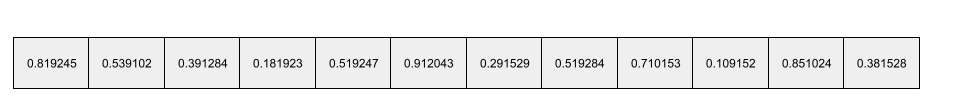An embedding vector is not a bunch of random numbers. An embedding layer determines these values through training, similar to the way a neural network learns other weights during training. Each element of the array is a rating along some characteristic of a tree species. Which element represents which tree species' characteristic? That's very hard for humans to determine.

The mathematically remarkable part of an embedding vector is that similar items have similar sets of floating-point numbers. For example, similar tree species have a more similar set of floating-point numbers than dissimilar tree species. Redwoods and sequoias are related tree species, so they'll have a more similar set of floating-pointing numbers than redwoods and coconut palms. The numbers in the embedding vector will change each time you retrain the model, even if you retrain the model with identical input.

## empirical risk minimization (ERM)

Choosing the function that minimizes loss on the training set. Contrast with structural risk minimization.

## encoder

#language

In general, any ML system that converts from a raw, sparse, or external representation into a more processed, denser, or more internal representation.

Encoders are often a component of a larger model, where they are frequently paired with a decoder. Some Transformers pair encoders with decoders, though other Transformers use only the encoder or only the decoder.

Some systems use the encoder's output as the input to a classification or regression network.

In sequence-to-sequence tasks, an encoder takes an input sequence and returns an internal state (a vector). Then, the decoder uses that internal state to predict the next sequence.

Refer to Transformer for the definition of an encoder in the Transformer architecture.

## ensemble

A collection of models trained independently whose predictions are averaged or aggregated. In many cases, an ensemble produces better predictions than a single model. For example, a random forest is an ensemble built from multiple decision trees. Note that not all decision forests are ensembles.

## entropy

#df

In information theory, a description of how unpredictable a probability distribution is. Alternatively, entropy is also defined as how much information each example contains. A distribution has the highest possible entropy when all values of a random variable are equally likely.

The entropy of a set with two possible values "0" and "1" (for example, the labels in a binary classification problem) has the following formula:

H = -p log p - q log q = -p log p - (1-p) * log (1-p)

where:

• H is the entropy.
• p is the fraction of "1" examples.
• q is the fraction of "0" examples. Note that q = (1 - p)
• log is generally log2. In this case, the entropy unit is a bit.

For example, suppose the following:

• 100 examples contain the value "1"
• 300 examples contain the value "0"

Therefore, the entropy value is:

• p = 0.25
• q = 0.75
• H = (-0.25)log2(0.25) - (0.75)log2(0.75) = 0.81 bits per example

A set that is perfectly balanced (for example, 200 "0"s and 200 "1"s) would have an entropy of 1.0 bit per example. As a set becomes more imbalanced, its entropy moves towards 0.0.

In decision trees, entropy helps formulate information gain to help the splitter select the conditions during the growth of a classification decision tree.

Compare entropy with:

Entropy is often called Shannon's entropy.

## environment

#rl

In reinforcement learning, the world that contains the agent and allows the agent to observe that world's state. For example, the represented world can be a game like chess, or a physical world like a maze. When the agent applies an action to the environment, then the environment transitions between states.

## episode

#rl

In reinforcement learning, each of the repeated attempts by the agent to learn an environment.

## epoch

#fundamentals

A full training pass over the entire training set such that each example has been processed once.

An epoch represents N/batch size training iterations, where N is the total number of examples.

For instance, suppose the following:

• The dataset consists of 1,000 examples.
• The batch size is 50 examples.

Therefore, a single epoch requires 20 iterations:

1 epoch = (N/batch size) = (1,000 / 50) = 20 iterations


## epsilon greedy policy

#rl

In reinforcement learning, a policy that either follows a random policy with epsilon probability or a greedy policy otherwise. For example, if epsilon is 0.9, then the policy follows a random policy 90% of the time and a greedy policy 10% of the time.

Over successive episodes, the algorithm reduces epsilon’s value in order to shift from following a random policy to following a greedy policy. By shifting the policy, the agent first randomly explores the environment and then greedily exploits the results of random exploration.

## equality of opportunity

#fairness
A fairness metric that checks whether, for a preferred label (one that confers an advantage or benefit to a person) and a given attribute, a classifier predicts that preferred label equally well for all values of that attribute. In other words, equality of opportunity measures whether the people who should qualify for an opportunity are equally likely to do so regardless of their group membership.

For example, suppose Glubbdubdrib University admits both Lilliputians and Brobdingnagians to a rigorous mathematics program. Lilliputians’ secondary schools offer a robust curriculum of math classes, and the vast majority of students are qualified for the university program. Brobdingnagians’ secondary schools don’t offer math classes at all, and as a result, far fewer of their students are qualified. Equality of opportunity is satisfied for the preferred label of "admitted" with respect to nationality (Lilliputian or Brobdingnagian) if qualified students are equally likely to be admitted irrespective of whether they're a Lilliputian or a Brobdingnagian.

For example, let's say 100 Lilliputians and 100 Brobdingnagians apply to Glubbdubdrib University, and admissions decisions are made as follows:

Table 1. Lilliputian applicants (90% are qualified)

Qualified Unqualified
Rejected 45 7
Total 90 10
Percentage of qualified students admitted: 45/90 = 50%
Percentage of unqualified students rejected: 7/10 = 70%
Total percentage of Lilliputian students admitted: (45+3)/100 = 48%

Table 2. Brobdingnagian applicants (10% are qualified):

Qualified Unqualified
Rejected 5 81
Total 10 90
Percentage of qualified students admitted: 5/10 = 50%
Percentage of unqualified students rejected: 81/90 = 90%
Total percentage of Brobdingnagian students admitted: (5+9)/100 = 14%

The preceding examples satisfy equality of opportunity for acceptance of qualified students because qualified Lilliputians and Brobdingnagians both have a 50% chance of being admitted.

See "Equality of Opportunity in Supervised Learning" for a more detailed discussion of equality of opportunity. Also see "Attacking discrimination with smarter machine learning" for a visualization exploring the tradeoffs when optimizing for equality of opportunity.

## equalized odds

#fairness
A fairness metric that checks if, for any particular label and attribute, a classifier predicts that label equally well for all values of that attribute.

For example, suppose Glubbdubdrib University admits both Lilliputians and Brobdingnagians to a rigorous mathematics program. Lilliputians' secondary schools offer a robust curriculum of math classes, and the vast majority of students are qualified for the university program. Brobdingnagians' secondary schools don’t offer math classes at all, and as a result, far fewer of their students are qualified. Equalized odds is satisfied provided that no matter whether an applicant is a Lilliputian or a Brobdingnagian, if they are qualified, they are equally as likely to get admitted to the program, and if they are not qualified, they are equally as likely to get rejected.

Let’s say 100 Lilliputians and 100 Brobdingnagians apply to Glubbdubdrib University, and admissions decisions are made as follows:

Table 3. Lilliputian applicants (90% are qualified)

Qualified Unqualified
Rejected 45 8
Total 90 10
Percentage of qualified students admitted: 45/90 = 50%
Percentage of unqualified students rejected: 8/10 = 80%
Total percentage of Lilliputian students admitted: (45+2)/100 = 47%

Table 4. Brobdingnagian applicants (10% are qualified):

Qualified Unqualified
Rejected 5 72
Total 10 90
Percentage of qualified students admitted: 5/10 = 50%
Percentage of unqualified students rejected: 72/90 = 80%
Total percentage of Brobdingnagian students admitted: (5+18)/100 = 23%

Equalized odds is satisfied because qualified Lilliputian and Brobdingnagian students both have a 50% chance of being admitted, and unqualified Lilliputian and Brobdingnagian have an 80% chance of being rejected.

Equalized odds is formally defined in "Equality of Opportunity in Supervised Learning" as follows: "predictor Ŷ satisfies equalized odds with respect to protected attribute A and outcome Y if Ŷ and A are independent, conditional on Y."

## Estimator

#TensorFlow

A deprecated TensorFlow API. Use tf.keras instead of Estimators.

## example

#fundamentals

The values of one row of features and possibly a label. Examples in supervised learning fall into two general categories:

• A labeled example consists of one or more features and a label. Labeled examples are used during training.
• An unlabeled example consists of one or more features but no label. Unlabeled examples are used during inference.

For instance, suppose you are training a model to determine the influence of weather conditions on student test scores. Here are three labeled examples:

Features Label
Temperature Humidity Pressure Test score
15 47 998 Good
19 34 1020 Excellent
18 92 1012 Poor

Here are three unlabeled examples:

Temperature Humidity Pressure
12 62 1014
21 47 1017
19 41 1021

The row of a dataset is typically the raw source for an example. That is, an example typically consists of a subset of the columns in the dataset. Furthermore, the features in an example can also include synthetic features, such as feature crosses.

## experience replay

#rl

In reinforcement learning, a DQN technique used to reduce temporal correlations in training data. The agent stores state transitions in a replay buffer, and then samples transitions from the replay buffer to create training data.

## experimenter's bias

#fairness

#seq

The tendency for gradients in deep neural networks (especially recurrent neural networks) to become surprisingly steep (high). Steep gradients often cause very large updates to the weights of each node in a deep neural network.

Models suffering from the exploding gradient problem become difficult or impossible to train. Gradient clipping can mitigate this problem.

## F1

A "roll-up" binary classification metric that relies on both precision and recall. Here is the formula:

$$F{_1} = \frac{\text{2 * precision * recall}} {\text{precision + recall}}$$

For example, given the following:

• precision = 0.6
• recall = 0.4
$$F{_1} = \frac{\text{2 * 0.6 * 0.4}} {\text{0.6 + 0.4}} = 0.48$$

When precision and recall are fairly similar (as in the preceding example), F1 is close to their mean. When precision and recall differ significantly, F1 is closer to the lower value. For example:

• precision = 0.9
• recall = 0.1
$$F{_1} = \frac{\text{2 * 0.9 * 0.1}} {\text{0.9 + 0.1}} = 0.18$$

## fairness constraint

#fairness
Applying a constraint to an algorithm to ensure one or more definitions of fairness are satisfied. Examples of fairness constraints include:

## fairness metric

#fairness

A mathematical definition of “fairness” that is measurable. Some commonly used fairness metrics include:

Many fairness metrics are mutually exclusive; see incompatibility of fairness metrics.

## false negative (FN)

#fundamentals

An example in which the model mistakenly predicts the negative class. For example, the model predicts that a particular email message is not spam (the negative class), but that email message actually is spam.

## false negative rate

The proportion of actual positive examples for which the model mistakenly predicted the negative class. The following formula calculates the false negative rate:

$$\text{false negative rate} = \frac{\text{false negatives}}{\text{false negatives} + \text{true positives}}$$

## false positive (FP)

#fundamentals

An example in which the model mistakenly predicts the positive class. For example, the model predicts that a particular email message is spam (the positive class), but that email message is actually not spam.

## false positive rate (FPR)

#fundamentals

The proportion of actual negative examples for which the model mistakenly predicted the positive class. The following formula calculates the false positive rate:

$$\text{false positive rate} = \frac{\text{false positives}}{\text{false positives} + \text{true negatives}}$$

The false positive rate is the x-axis in an ROC curve.

## feature

#fundamentals

An input variable to a machine learning model. An example consists of one or more features. For instance, suppose you are training a model to determine the influence of weather conditions on student test scores. The following table shows three examples, each of which contains three features and one label:

Features Label
Temperature Humidity Pressure Test score
15 47 998 92
19 34 1020 84
18 92 1012 87

Contrast with label.

## feature cross

#fundamentals

A synthetic feature formed by "crossing" categorical or bucketed features.

For example, consider a "mood forecasting" model that represents temperature in one of the following four buckets:

• freezing
• chilly
• temperate
• warm

And represents wind speed in one of the following three buckets:

• still
• light
• windy

Without feature crosses, the linear model trains independently on each of the preceding seven various buckets. So, the model trains on, for instance, freezing independently of the training on, for instance, windy.

Alternatively, you could create a feature cross of temperature and wind speed. This synthetic feature would have the following 12 possible values:

• freezing-still
• freezing-light
• freezing-windy
• chilly-still
• chilly-light
• chilly-windy
• temperate-still
• temperate-light
• temperate-windy
• warm-still
• warm-light
• warm-windy

Thanks to feature crosses, the model can learn mood differences between a freezing-windy day and a freezing-still day.

If you create a synthetic feature from two features that each have a lot of different buckets, the resulting feature cross will have a huge number of possible combinations. For example, if one feature has 1,000 buckets and the other feature has 2,000 buckets, the resulting feature cross has 2,000,000 buckets.

Formally, a cross is a Cartesian product.

Feature crosses are mostly used with linear models and are rarely used with neural networks.

## feature engineering

#fundamentals
#TensorFlow

A process that involves the following steps:

1. Determining which features might be useful in training a model.
2. Converting raw data from the dataset into efficient versions of those features.

For example, you might determine that temperature might be a useful feature. Then, you might experiment with bucketing to optimize what the model can learn from different temperature ranges.

Feature engineering is sometimes called feature extraction.

## feature extraction

Overloaded term having either of the following definitions:

## feature importances

#df

Synonym for variable importances.

## feature set

#fundamentals

The group of features your machine learning model trains on. For example, postal code, property size, and property condition might comprise a simple feature set for a model that predicts housing prices.

## feature spec

#TensorFlow

Describes the information required to extract features data from the tf.Example protocol buffer. Because the tf.Example protocol buffer is just a container for data, you must specify the following:

• the data to extract (that is, the keys for the features)
• the data type (for example, float or int)
• The length (fixed or variable)

## feature vector

#fundamentals

The array of feature values comprising an example. The feature vector is input during training and during inference. For example, the feature vector for a model with two discrete features might be:

[0.92, 0.56]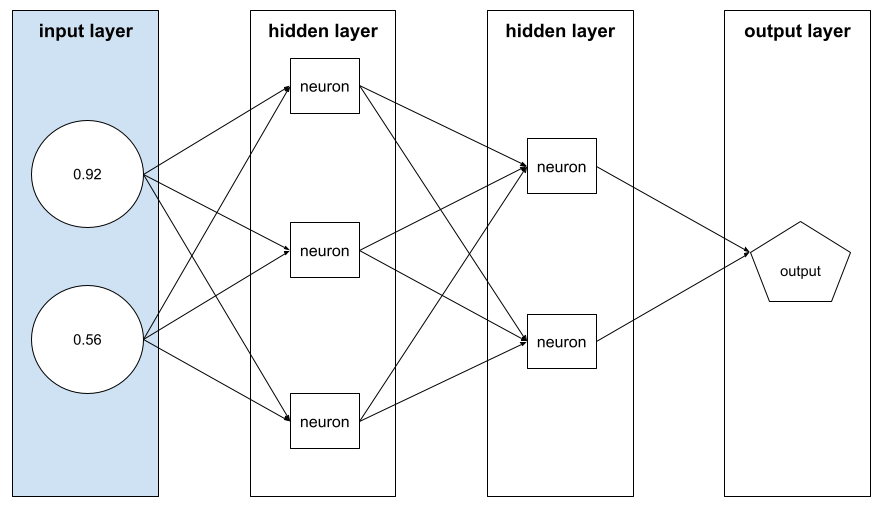Each example supplies different values for the feature vector, so the feature vector for the next example could be something like:

[0.73, 0.49]


Feature engineering determines how to represent features in the feature vector. For example, a binary categorical feature with five possible values might be represented with one-hot encoding. In this case, the portion of the feature vector for a particular example would consist of four zeroes and a single 1.0 in the third position, as follows:

[0.0, 0.0, 1.0, 0.0, 0.0]


As another example, suppose your model consists of three features:

• a binary categorical feature with five possible values represented with one-hot encoding; for example: [0.0, 1.0, 0.0, 0.0, 0.0]
• another binary categorical feature with three possible values represented with one-hot encoding; for example: [0.0, 0.0, 1.0]
• a floating-point feature; for example: 8.3.

In this case, the feature vector for each example would be represented by nine values. Given the example values in the preceding list, the feature vector would be:

0.0
1.0
0.0
0.0
0.0
0.0
0.0
1.0
8.3


## federated learning

A distributed machine learning approach that trains machine learning models using decentralized examples residing on devices such as smartphones. In federated learning, a subset of devices downloads the current model from a central coordinating server. The devices use the examples stored on the devices to make improvements to the model. The devices then upload the model improvements (but not the training examples) to the coordinating server, where they are aggregated with other updates to yield an improved global model. After the aggregation, the model updates computed by devices are no longer needed, and can be discarded.

Since the training examples are never uploaded, federated learning follows the privacy principles of focused data collection and data minimization.

## feedback loop

#fundamentals

In machine learning, a situation in which a model's predictions influence the training data for the same model or another model. For example, a model that recommends movies will influence the movies that people see, which will then influence subsequent movie recommendation models.

## feedforward neural network (FFN)

A neural network without cyclic or recursive connections. For example, traditional deep neural networks are feedforward neural networks. Contrast with recurrent neural networks, which are cyclic.

## few-shot learning

A machine learning approach, often used for object classification, designed to train effective classifiers from only a small number of training examples.

## few-shot prompting

#language
#generativeAI

A prompt that contains more than one (a "few") example demonstrating how the large language model should respond. For example, the following lengthy prompt contains two examples showing a large language model how to answer a query.

Parts of one prompt Notes
What is the official currency of the specified country? The question you want the LLM to answer.
France: EUR One example.
United Kingdom: GBP Another example.
India: The actual query.

Few-shot prompting generally produces more desirable results than zero-shot prompting and one-shot prompting. However, few-shot prompting requires a lengthier prompt.

Few-shot prompting is a form of few-shot learning applied to prompt-based learning.

## Fiddle

#language

A Python-first configuration library that sets the values of functions and classes without invasive code or infrastructure. In the case of Pax—and other ML codebases—these functions and classes represent models and training hyperparameters.

Fiddle assumes that machine learning codebases are typically divided into:

• Library code, which defines the layers and optimizers.
• Dataset "glue" code, which calls the libraries and wires everything together.

Fiddle captures the call structure of the glue code in an unevaluated and mutable form.

## fine tuning

#language
#image
#generativeAI

A second, task-specific training pass performed on a pre-trained model to refine its parameters for a specific use case. For example, the full training sequence for some large language models is as follows:

1. Pre-training: Train a large language model on a vast general dataset, such as all the English language Wikipedia pages.
2. Fine-tuning: Train the pre-trained model to perform a specific task, such as responding to medical queries. Fine-tuning typically involves hundreds or thousands of examples focused on the specific task.

As another example, the full training sequence for a large image model is as follows:

1. Pre-training: Train a large image model on a vast general image dataset, such as all the images in Wikimedia commons.
2. Fine-tuning: Train the pre-trained model to perform a specific task, such as generating images of orcas.

Fine-tuning can entail any combination of the following strategies:

• Modifying all of the pre-trained model's existing parameters. This is sometimes called full fine-tuning.
• Modifying only some of the pre-trained model's existing parameters (typically, the layers closest to the output layer), while keeping other existing parameters unchanged (typically, the layers closest to the input layer). See parameter-efficient tuning.
• Adding more layers, typically on top of the existing layers closest to the output layer.

Fine-tuning is a form of transfer learning. As such, fine-tuning might use a different loss function or a different model type than those used to train the pre-trained model. For example, you could fine-tune a pre-trained large image model to produce a regression model that returns the number of birds in an input image.

Compare and contrast fine-tuning with the following terms:

## Flax

#language

A high-performance open-source library for deep learning built on top of JAX. Flax provides functions for training neural networks, as well as methods for evaluating their performance.

## Flaxformer

#language

An open-source Transformer library, built on Flax, designed primarily for natural language processing and multimodal research.

## forget gate

#seq

The portion of a Long Short-Term Memory cell that regulates the flow of information through the cell. Forget gates maintain context by deciding which information to discard from the cell state.

## full softmax

Synonym for softmax.

Contrast with candidate sampling.

## fully connected layer

A hidden layer in which each node is connected to every node in the subsequent hidden layer.

A fully connected layer is also known as a dense layer.

## generalization

#fundamentals

A model's ability to make correct predictions on new, previously unseen data. A model that can generalize is the opposite of a model that is overfitting.

## generalization curve

#fundamentals

A plot of both training loss and validation loss as a function of the number of iterations.

A generalization curve can help you detect possible overfitting. For example, the following generalization curve suggests overfitting because validation loss ultimately becomes significantly higher than training loss.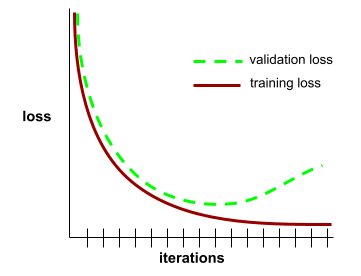## generalized linear model

A generalization of least squares regression models, which are based on Gaussian noise, to other types of models based on other types of noise, such as Poisson noise or categorical noise. Examples of generalized linear models include:

The parameters of a generalized linear model can be found through convex optimization.

Generalized linear models exhibit the following properties:

• The average prediction of the optimal least squares regression model is equal to the average label on the training data.
• The average probability predicted by the optimal logistic regression model is equal to the average label on the training data.

The power of a generalized linear model is limited by its features. Unlike a deep model, a generalized linear model cannot "learn new features."

A system to create new data in which a generator creates data and a discriminator determines whether that created data is valid or invalid.

## generative AI

#language
#image
#generativeAI

An emerging transformative field with no formal definition. That said, most experts agree that generative AI models can create ("generate") content that is all of the following:

• complex
• coherent
• original

For example, a generative AI model can create sophisticated essays or images.

Some earlier technologies, including LSTMs and RNNs, can also generate original and coherent content. Some experts view these earlier technologies as generative AI, while others feel that true generative AI requires more complex output than those earlier technologies can produce.

Contrast with predictive ML.

## generative model

Practically speaking, a model that does either of the following:

• Creates (generates) new examples from the training dataset. For example, a generative model could create poetry after training on a dataset of poems. The generator part of a generative adversarial network falls into this category.
• Determines the probability that a new example comes from the training set, or was created from the same mechanism that created the training set. For example, after training on a dataset consisting of English sentences, a generative model could determine the probability that new input is a valid English sentence.

A generative model can theoretically discern the distribution of examples or particular features in a dataset. That is:

p(examples)


Unsupervised learning models are generative.

Contrast with discriminative models.

## generator

The subsystem within a generative adversarial network that creates new examples.

Contrast with discriminative model.

## gini impurity

#df

A metric similar to entropy. Splitters use values derived from either gini impurity or entropy to compose conditions for classification decision trees. Information gain is derived from entropy. There is no universally accepted equivalent term for the metric derived from gini impurity; however, this unnamed metric is just as important as information gain.

Gini impurity is also called gini index, or simply gini.

## GPT (Generative Pre-trained Transformer)

#language

A family of Transformer-based large language models developed by OpenAI.

GPT variants can apply to multiple modalities, including:

• image generation (for example, ImageGPT)
• text-to-image generation (for example, DALL-E).

The vector of partial derivatives with respect to all of the independent variables. In machine learning, the gradient is the vector of partial derivatives of the model function. The gradient points in the direction of steepest ascent.

## gradient boosted (decision) trees (GBT)

#df

A type of decision forest in which:

#df

A training algorithm where weak models are trained to iteratively improve the quality (reduce the loss) of a strong model. For example, a weak model could be a linear or small decision tree model. The strong model becomes the sum of all the previously trained weak models.

In the simplest form of gradient boosting, at each iteration, a weak model is trained to predict the loss gradient of the strong model. Then, the strong model's output is updated by subtracting the predicted gradient, similar to gradient descent.

$$F_{0} = 0$$ $$F_{i+1} = F_i - \xi f_i$$

where:

• $F_{0}$ is the starting strong model.
• $F_{i+1}$ is the next strong model.
• $F_{i}$ is the current strong model.
• $\xi$ is a value between 0.0 and 1.0 called shrinkage, which is analogous to the learning rate in gradient descent.
• $f_{i}$ is the weak model trained to predict the loss gradient of $F_{i}$.

Modern variations of gradient boosting also include the second derivative (Hessian) of the loss in their computation.

Decision trees are commonly used as weak models in gradient boosting. See gradient boosted (decision) trees.

#seq

A commonly used mechanism to mitigate the exploding gradient problem by artificially limiting (clipping) the maximum value of gradients when using gradient descent to train a model.

#fundamentals

A mathematical technique to minimize loss. Gradient descent iteratively adjusts weights and biases, gradually finding the best combination to minimize loss.

Gradient descent is older—much, much older—than machine learning.

## graph

#TensorFlow

In TensorFlow, a computation specification. Nodes in the graph represent operations. Edges are directed and represent passing the result of an operation (a Tensor) as an operand to another operation. Use TensorBoard to visualize a graph.

## graph execution

#TensorFlow

A TensorFlow programming environment in which the program first constructs a graph and then executes all or part of that graph. Graph execution is the default execution mode in TensorFlow 1.x.

Contrast with eager execution.

## greedy policy

#rl

In reinforcement learning, a policy that always chooses the action with the highest expected return.

## ground truth

#fundamentals

Reality.

The thing that actually happened.

For example, consider a binary classification model that predicts whether a student in their first year of university will graduate within six years. Ground truth for this model is whether or not that student actually graduated within six years.

#fairness

Assuming that what is true for an individual is also true for everyone in that group. The effects of group attribution bias can be exacerbated if a convenience sampling is used for data collection. In a non-representative sample, attributions may be made that do not reflect reality.

## hallucination

#language

The production of plausible-seeming but factually incorrect output by a generative AI model that purports to be making an assertion about the real world. For example, a generative AI model that claims that Barack Obama died in 1865 is hallucinating.

## hashing

In machine learning, a mechanism for bucketing categorical data, particularly when the number of categories is large, but the number of categories actually appearing in the dataset is comparatively small.

For example, Earth is home to about 73,000 tree species. You could represent each of the 73,000 tree species in 73,000 separate categorical buckets. Alternatively, if only 200 of those tree species actually appear in a dataset, you could use hashing to divide tree species into perhaps 500 buckets.

A single bucket could contain multiple tree species. For example, hashing could place baobab and red maple—two genetically dissimilar species—into the same bucket. Regardless, hashing is still a good way to map large categorical sets into the desired number of buckets. Hashing turns a categorical feature having a large number of possible values into a much smaller number of values by grouping values in a deterministic way.

## heuristic

A simple and quickly implemented solution to a problem. For example, "With a heuristic, we achieved 86% accuracy. When we switched to a deep neural network, accuracy went up to 98%."

## hidden layer

#fundamentals

A layer in a neural network between the input layer (the features) and the output layer (the prediction). Each hidden layer consists of one or more neurons. For example, the following neural network contains two hidden layers, the first with three neurons and the second with two neurons: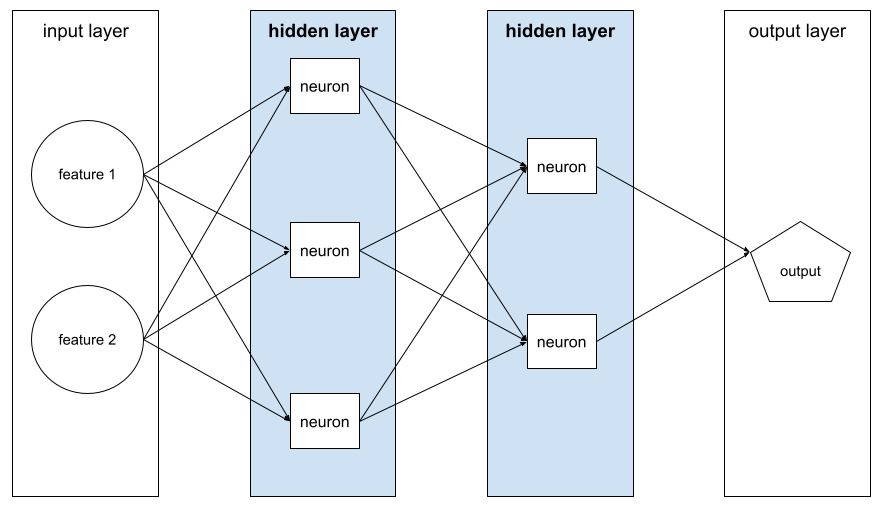A deep neural network contains more than one hidden layer. For example, the preceding illustration is a deep neural network because the model contains two hidden layers.

## hierarchical clustering

#clustering

A category of clustering algorithms that create a tree of clusters. Hierarchical clustering is well-suited to hierarchical data, such as botanical taxonomies. There are two types of hierarchical clustering algorithms:

• Agglomerative clustering first assigns every example to its own cluster, and iteratively merges the closest clusters to create a hierarchical tree.
• Divisive clustering first groups all examples into one cluster and then iteratively divides the cluster into a hierarchical tree.

Contrast with centroid-based clustering.

## hinge loss

A family of loss functions for classification designed to find the decision boundary as distant as possible from each training example, thus maximizing the margin between examples and the boundary. KSVMs use hinge loss (or a related function, such as squared hinge loss). For binary classification, the hinge loss function is defined as follows:

$$\text{loss} = \text{max}(0, 1 - (y * y'))$$

where y is the true label, either -1 or +1, and y' is the raw output of the classifier model:

$$y' = b + w_1x_1 + w_2x_2 + … w_nx_n$$

Consequently, a plot of hinge loss vs. (y * y') looks as follows:## holdout data

Examples intentionally not used ("held out") during training. The validation dataset and test dataset are examples of holdout data. Holdout data helps evaluate your model's ability to generalize to data other than the data it was trained on. The loss on the holdout set provides a better estimate of the loss on an unseen dataset than does the loss on the training set.

## host

#TensorFlow

When training an ML model on accelerator chips (GPUs or TPUs), the part of the system that controls both of the following:

• The overall flow of the code.
• The extraction and transformation of the input pipeline.

The host typically runs on a CPU, not on an accelerator chip; the device manipulates tensors on the accelerator chips.

## hyperparameter

#fundamentals

The variables that you or a hyperparameter tuning service adjust during successive runs of training a model. For example, learning rate is a hyperparameter. You could set the learning rate to 0.01 before one training session. If you determine that 0.01 is too high, you could perhaps set the learning rate to 0.003 for the next training session.

In contrast, parameters are the various weights and bias that the model learns during training.

## hyperplane

A boundary that separates a space into two subspaces. For example, a line is a hyperplane in two dimensions and a plane is a hyperplane in three dimensions. More typically in machine learning, a hyperplane is the boundary separating a high-dimensional space. Kernel Support Vector Machines use hyperplanes to separate positive classes from negative classes, often in a very high-dimensional space.

## i.i.d.

Abbreviation for independently and identically distributed.

## image recognition

#image

A process that classifies object(s), pattern(s), or concept(s) in an image. Image recognition is also known as image classification.

## imbalanced dataset

Synonym for class-imbalanced dataset.

## implicit bias

#fairness

Automatically making an association or assumption based on one’s mental models and memories. Implicit bias can affect the following:

• How data is collected and classified.
• How machine learning systems are designed and developed.

For example, when building a classifier to identify wedding photos, an engineer may use the presence of a white dress in a photo as a feature. However, white dresses have been customary only during certain eras and in certain cultures.

## imputation

Short form of value imputation.

## incompatibility of fairness metrics

#fairness

The idea that some notions of fairness are mutually incompatible and cannot be satisfied simultaneously. As a result, there is no single universal metric for quantifying fairness that can be applied to all ML problems.

While this may seem discouraging, incompatibility of fairness metrics doesn’t imply that fairness efforts are fruitless. Instead, it suggests that fairness must be defined contextually for a given ML problem, with the goal of preventing harms specific to its use cases.

See "On the (im)possibility of fairness" for a more detailed discussion of this topic.

## in-context learning

#language
#generativeAI

Synonym for few-shot prompting.

## independently and identically distributed (i.i.d)

#fundamentals

Data drawn from a distribution that doesn't change, and where each value drawn doesn't depend on values that have been drawn previously. An i.i.d. is the ideal gas of machine learning—a useful mathematical construct but almost never exactly found in the real world. For example, the distribution of visitors to a web page may be i.i.d. over a brief window of time; that is, the distribution doesn't change during that brief window and one person's visit is generally independent of another's visit. However, if you expand that window of time, seasonal differences in the web page's visitors may appear.

## individual fairness

#fairness

A fairness metric that checks whether similar individuals are classified similarly. For example, Brobdingnagian Academy might want to satisfy individual fairness by ensuring that two students with identical grades and standardized test scores are equally likely to gain admission.

Note that individual fairness relies entirely on how you define "similarity" (in this case, grades and test scores), and you can run the risk of introducing new fairness problems if your similarity metric misses important information (such as the rigor of a student’s curriculum).

See "Fairness Through Awareness" for a more detailed discussion of individual fairness.

## inference

#fundamentals

In machine learning, the process of making predictions by applying a trained model to unlabeled examples.

Inference has a somewhat different meaning in statistics. See the Wikipedia article on statistical inference for details.

## inference path

#df

In a decision tree, during inference, the route a particular example takes from the root to other conditions, terminating with a leaf. For instance, in the following decision tree, the thicker arrows show the inference path for an example with the following feature values:

• x = 7
• y = 12
• z = -3

The inference path in the following illustration travels through three conditions before reaching the leaf (Zeta).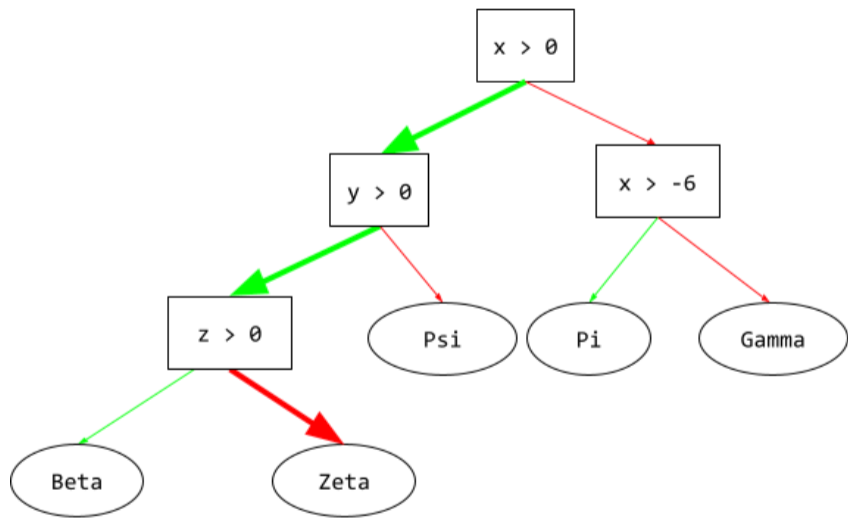The three thick arrows show the inference path.

## information gain

#df

In decision forests, the difference between a node's entropy and the weighted (by number of examples) sum of the entropy of its children nodes. A node's entropy is the entropy of the examples in that node.

For example, consider the following entropy values:

• entropy of parent node = 0.6
• entropy of one child node with 16 relevant examples = 0.2
• entropy of another child node with 24 relevant examples = 0.1

So 40% of the examples are in one child node and 60% are in the other child node. Therefore:

• weighted entropy sum of child nodes = (0.4 * 0.2) + (0.6 * 0.1) = 0.14

So, the information gain is:

• information gain = entropy of parent node - weighted entropy sum of child nodes
• information gain = 0.6 - 0.14 = 0.46

Most splitters seek to create conditions that maximize information gain.

## in-group bias

#fairness

Showing partiality to one's own group or own characteristics. If testers or raters consist of the machine learning developer's friends, family, or colleagues, then in-group bias may invalidate product testing or the dataset.

## input generator

A mechanism by which data is loaded into a neural network.

An input generator can be thought of as a component responsible for processing raw data into tensors which are iterated over to generate batches for training, evaluation, and inference.

## input layer

#fundamentals

The layer of a neural network that holds the feature vector. That is, the input layer provides examples for training or inference. For example, the input layer in the following neural network consists of two features: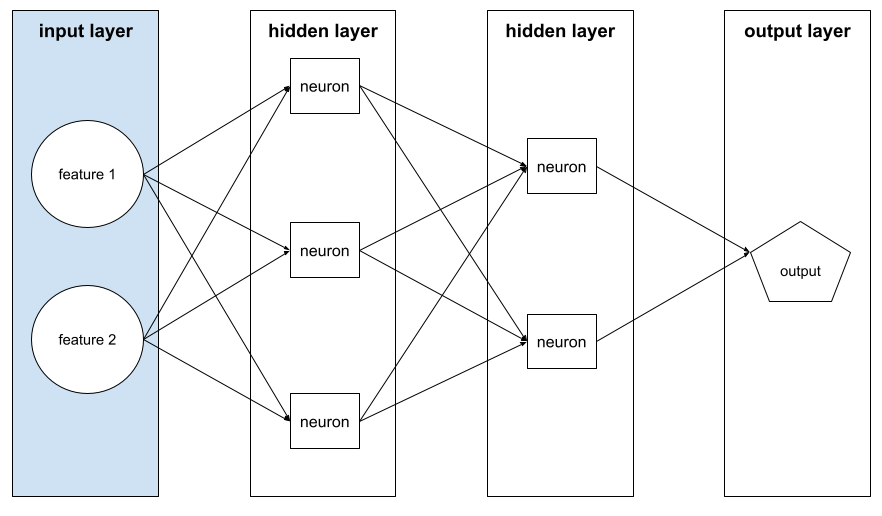## in-set condition

#df

In a decision tree, a condition that tests for the presence of one item in a set of items. For example, the following is an in-set condition:

  house-style in [tudor, colonial, cape]


During inference, if the value of the house-style feature is tudor or colonial or cape, then this condition evaluates to Yes. If the value of the house-style feature is something else (for example, ranch), then this condition evaluates to No.

In-set conditions usually lead to more efficient decision trees than conditions that test one-hot encoded features.

## instance

Synonym for example.

## instruction tuning

#generativeAI

A form of fine-tuning that improves a generative AI model's ability to follow instructions. Instruction tuning involves training a model on a series of instructions prompts, typically covering a wide variety of tasks. The resulting instruction-tuned model then tends to generate useful responses to zero-shot prompts across a variety of tasks.

Compare and contrast with:

## interpretability

#fundamentals

The ability to explain or to present an ML model's reasoning in understandable terms to a human.

Most linear regression models, for example, are highly interpretable. (You merely need to look at the trained weights for each feature.) Decision forests are also highly interpretable. Some models, however, require sophisticated visualization to become interpretable.

You can use the Learning Interpretability Tool (LIT) to interpret ML models.

## inter-rater agreement

A measurement of how often human raters agree when doing a task. If raters disagree, the task instructions may need to be improved. Also sometimes called inter-annotator agreement or inter-rater reliability. See also Cohen's kappa, which is one of the most popular inter-rater agreement measurements.

## intersection over union (IoU)

#image

The intersection of two sets divided by their union. In machine-learning image-detection tasks, IoU is used to measure the accuracy of the model’s predicted bounding box with respect to the ground-truth bounding box. In this case, the IoU for the two boxes is the ratio between the overlapping area and the total area, and its value ranges from 0 (no overlap of predicted bounding box and ground-truth bounding box) to 1 (predicted bounding box and ground-truth bounding box have the exact same coordinates).

For example, in the image below:

• The predicted bounding box (the coordinates delimiting where the model predicts the night table in the painting is located) is outlined in purple.
• The ground-truth bounding box (the coordinates delimiting where the night table in the painting is actually located) is outlined in green.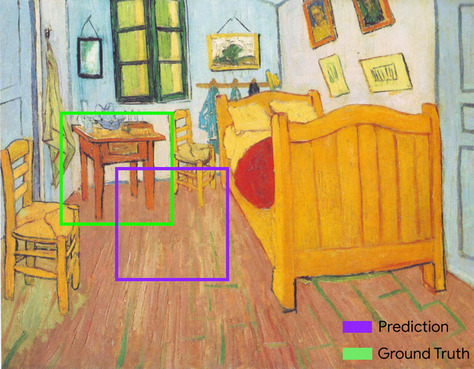Here, the intersection of the bounding boxes for prediction and ground truth (below left) is 1, and the union of the bounding boxes for prediction and ground truth (below right) is 7, so the IoU is $$\frac{1}{7}$$.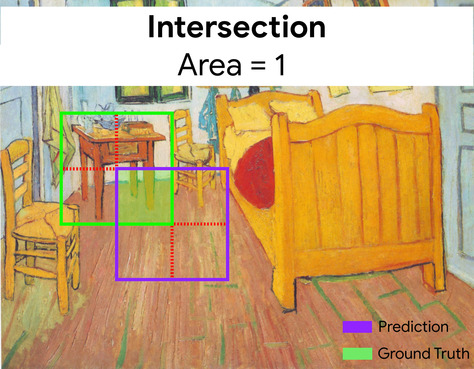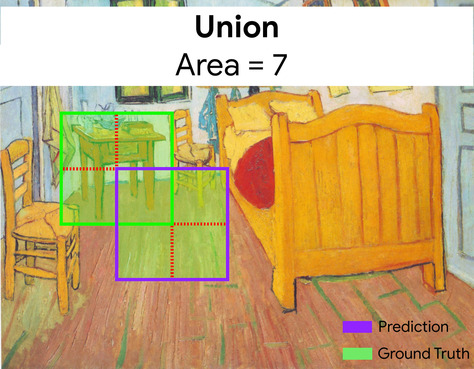## IoU

Abbreviation for intersection over union.

## item matrix

#recsystems

In recommendation systems, a matrix of embedding vectors generated by matrix factorization that holds latent signals about each item. Each row of the item matrix holds the value of a single latent feature for all items. For example, consider a movie recommendation system. Each column in the item matrix represents a single movie. The latent signals might represent genres, or might be harder-to-interpret signals that involve complex interactions among genre, stars, movie age, or other factors.

The item matrix has the same number of columns as the target matrix that is being factorized. For example, given a movie recommendation system that evaluates 10,000 movie titles, the item matrix will have 10,000 columns.

## items

#recsystems

In a recommendation system, the entities that a system recommends. For example, videos are the items that a video store recommends, while books are the items that a bookstore recommends.

## iteration

#fundamentals

A single update of a model's parameters—the model's weights and biases—during training. The batch size determines how many examples the model processes in a single iteration. For instance, if the batch size is 20, then the model processes 20 examples before adjusting the parameters.

When training a neural network, a single iteration involves the following two passes:

1. A forward pass to evaluate loss on a single batch.
2. A backward pass (backpropagation) to adjust the model's parameters based on the loss and the learning rate.

## JAX

An array computing library, bringing together XLA (Accelerated Linear Algebra) and automatic differentiation for high-performance numerical computing. JAX provides a simple and powerful API for writing accelerated numerical code with composable transformations. JAX provides features such as:

• grad (automatic differentiation)
• jit (just-in-time compilation)
• vmap (automatic vectorization or batching)
• pmap (parallelization)

JAX is a language for expressing and composing transformations of numerical code, analogous—but much larger in scope—to Python’s NumPy library. (In fact, the .numpy library under JAX is a functionally equivalent, but entirely rewritten version of the Python NumPy library.)

JAX is particularly well-suited for speeding up many machine learning tasks by transforming the models and data into a form suitable for parallelism across GPU and TPU accelerator chips.

Flax, Optax, Pax, and many other libraries are built on the JAX infrastructure.

## Keras

A popular Python machine learning API. Keras runs on several deep learning frameworks, including TensorFlow, where it is made available as tf.keras.

## Kernel Support Vector Machines (KSVMs)

A classification algorithm that seeks to maximize the margin between positive and negative classes by mapping input data vectors to a higher dimensional space. For example, consider a classification problem in which the input dataset has a hundred features. To maximize the margin between positive and negative classes, a KSVM could internally map those features into a million-dimension space. KSVMs uses a loss function called hinge loss.

## keypoints

#image

The coordinates of particular features in an image. For example, for an image recognition model that distinguishes flower species, keypoints might be the center of each petal, the stem, the stamen, and so on.

## k-fold cross validation

An algorithm for predicting a model's ability to generalize to new data. The k in k-fold refers to the number of equal groups you divide a dataset's examples into; that is, you train and test your model k times. For each round of training and testing, a different group is the test set, and all remaining groups become the training set. After k rounds of training and testing, you calculate the mean and standard deviation of the desired test metric(s).

For example, suppose your dataset consists of 120 examples. Further suppose, you decide to set k to 4. Therefore, after shuffling the examples, you divide the dataset into four equal groups of 30 examples and conduct four training/testing rounds: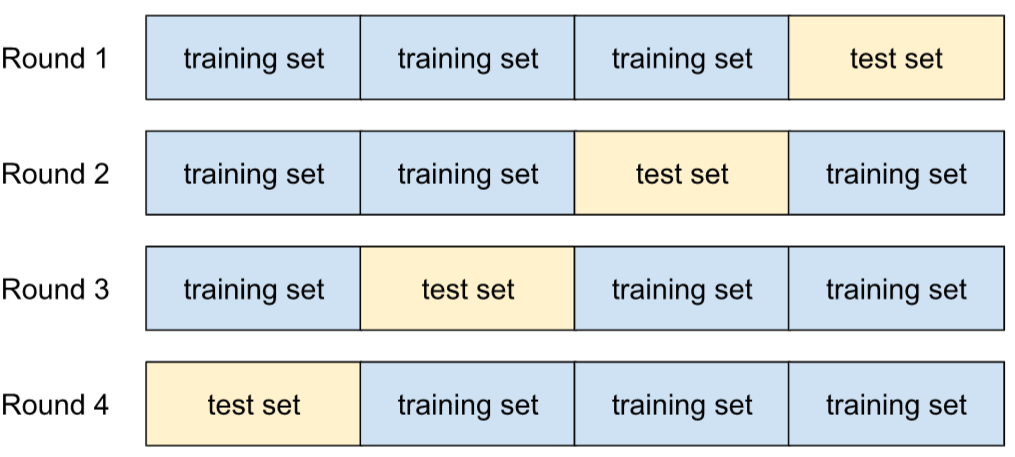For example, Mean Squared Error (MSE) might be the most meaningful metric for a linear regression model. Therefore, you would find the mean and standard deviation of the MSE across all four rounds.

## k-means

#clustering

A popular clustering algorithm that groups examples in unsupervised learning. The k-means algorithm basically does the following:

• Iteratively determines the best k center points (known as centroids).
• Assigns each example to the closest centroid. Those examples nearest the same centroid belong to the same group.

The k-means algorithm picks centroid locations to minimize the cumulative square of the distances from each example to its closest centroid.

For example, consider the following plot of dog height to dog width:If k=3, the k-means algorithm will determine three centroids. Each example is assigned to its closest centroid, yielding three groups:Imagine that a manufacturer wants to determine the ideal sizes for small, medium, and large sweaters for dogs. The three centroids identify the mean height and mean width of each dog in that cluster. So, the manufacturer should probably base sweater sizes on those three centroids. Note that the centroid of a cluster is typically not an example in the cluster.

The preceding illustrations shows k-means for examples with only two features (height and width). Note that k-means can group examples across many features.

## k-median

#clustering

A clustering algorithm closely related to k-means. The practical difference between the two is as follows:

• In k-means, centroids are determined by minimizing the sum of the squares of the distance between a centroid candidate and each of its examples.
• In k-median, centroids are determined by minimizing the sum of the distance between a centroid candidate and each of its examples.

Note that the definitions of distance are also different:

• k-means relies on the Euclidean distance from the centroid to an example. (In two dimensions, the Euclidean distance means using the Pythagorean theorem to calculate the hypotenuse.) For example, the k-means distance between (2,2) and (5,-2) would be:
$${\text{Euclidean distance}} = {\sqrt {(2-5)^2 + (2--2)^2}} = 5$$
• k-median relies on the Manhattan distance from the centroid to an example. This distance is the sum of the absolute deltas in each dimension. For example, the k-median distance between (2,2) and (5,-2) would be:
$${\text{Manhattan distance}} = \lvert 2-5 \rvert + \lvert 2--2 \rvert = 7$$

## L0 regularization

#fundamentals

A type of regularization that penalizes the total number of nonzero weights in a model. For example, a model having 11 nonzero weights would be penalized more than a similar model having 10 nonzero weights.

L0 regularization is sometimes called L0-norm regularization.

## L1 loss

#fundamentals

A loss function that calculates the absolute value of the difference between actual label values and the values that a model predicts. For example, here's the calculation of L1 loss for a batch of five examples:

Actual value of example Model's predicted value Absolute value of delta
7 6 1
5 4 1
8 11 3
4 6 2
9 8 1
8 = L1 loss

L1 loss is less sensitive to outliers than L2 loss.

The Mean Absolute Error is the average L1 loss per example.

## L1 regularization

#fundamentals

A type of regularization that penalizes weights in proportion to the sum of the absolute value of the weights. L1 regularization helps drive the weights of irrelevant or barely relevant features to exactly 0. A feature with a weight of 0 is effectively removed from the model.

Contrast with L2 regularization.

## L2 loss

#fundamentals

A loss function that calculates the square of the difference between actual label values and the values that a model predicts. For example, here's the calculation of L2 loss for a batch of five examples:

Actual value of example Model's predicted value Square of delta
7 6 1
5 4 1
8 11 9
4 6 4
9 8 1
16 = L2 loss

Due to squaring, L2 loss amplifies the influence of outliers. That is, L2 loss reacts more strongly to bad predictions than L1 loss. For example, the L1 loss for the preceding batch would be 8 rather than 16. Notice that a single outlier accounts for 9 of the 16.

Regression models typically use L2 loss as the loss function.

The Mean Squared Error is the average L2 loss per example. Squared loss is another name for L2 loss.

## L2 regularization

#fundamentals

A type of regularization that penalizes weights in proportion to the sum of the squares of the weights. L2 regularization helps drive outlier weights (those with high positive or low negative values) closer to 0 but not quite to 0. Features with values very close to 0 remain in the model but don't influence the model's prediction very much.

L2 regularization always improves generalization in linear models.

Contrast with L1 regularization.

## label

#fundamentals

In supervised machine learning, the "answer" or "result" portion of an example.

Each labeled example consists of one or more features and a label. For instance, in a spam detection dataset, the label would probably be either "spam" or "not spam." In a rainfall dataset, the label might be the amount of rain that fell during a certain period.

## labeled example

#fundamentals

An example that contains one or more features and a label. For example, the following table shows three labeled examples from a house valuation model, each with three features and one label:

Number of bedrooms Number of bathrooms House age House price (label)
3 2 15 $345,000 2 1 72$179,000
4 2 34 \$392,000

In supervised machine learning, models train on labeled examples and make predictions on unlabeled examples.

Contrast labeled example with unlabeled examples.

## label leakage

A model design flaw in which a feature is a proxy for the label. For example, consider a binary classification model that predicts whether or not a prospective customer will purchase a particular product. Suppose that one of the features for the model is a Boolean named SpokeToCustomerAgent. Further suppose that a customer agent is only assigned after the prospective customer has actually purchased the product. During training, the model will quickly learn the association between SpokeToCustomerAgent and the label.

## lambda

#fundamentals

Synonym for regularization rate.

Lambda is an overloaded term. Here we're focusing on the term's definition within regularization.

## LaMDA (Language Model for Dialogue Applications)

#language

A Transformer-based large language model developed by Google trained on a large dialogue dataset that can generate realistic conversational responses.

LaMDA: our breakthrough conversation technology provides an overview.

## landmarks

#image

Synonym for keypoints.

## language model

#language

A model that estimates the probability of a token or sequence of tokens occurring in a longer sequence of tokens.

## large language model

#language

An informal term with no strict definition that usually means a language model that has a high number of parameters. Some large language models contain over 100 billion parameters.

## layer

#fundamentals

A set of neurons in a neural network. Three common types of layers are as follows:

For example, the following illustration shows a neural network with one input layer, two hidden layers, and one output layer: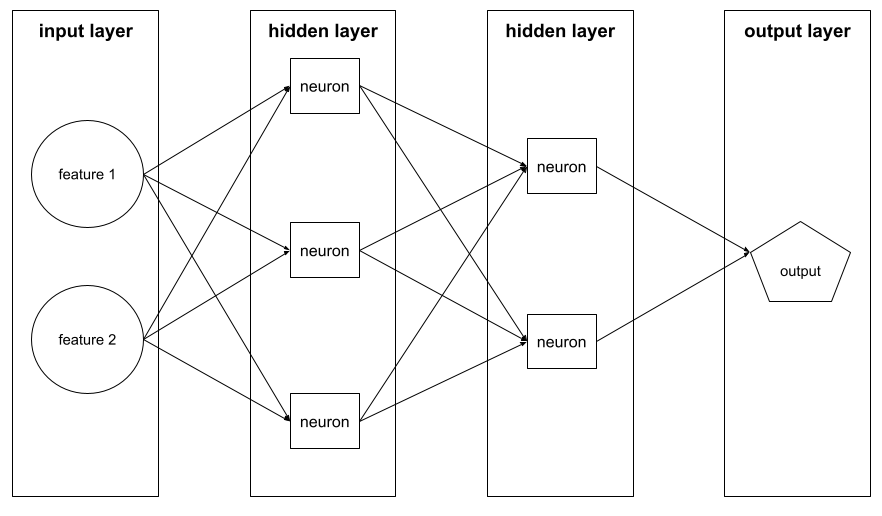In TensorFlow, layers are also Python functions that take Tensors and configuration options as input and produce other tensors as output.

## Layers API (tf.layers)

#TensorFlow

A TensorFlow API for constructing a deep neural network as a composition of layers. The Layers API enables you to build different types of layers, such as:

• tf.layers.Dense for a fully-connected layer.
• tf.layers.Conv2D for a convolutional layer.

The Layers API follows the Keras layers API conventions. That is, aside from a different prefix, all functions in the Layers API have the same names and signatures as their counterparts in the Keras layers API.

## leaf

#df

Any endpoint in a decision tree. Unlike a condition, a leaf doesn't perform a test. Rather, a leaf is a possible prediction. A leaf is also the terminal node of an inference path.

For example, the following decision tree contains three leaves: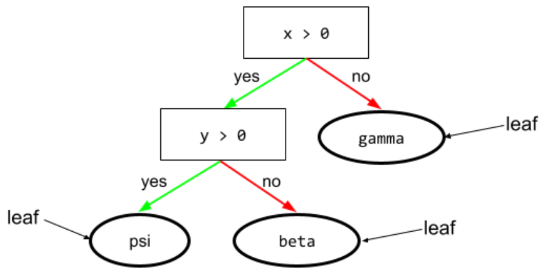## Learning Interpretability Tool (LIT)

A visual, interactive model-understanding and data visualization tool.

You can use open-source LIT to interpret models or to visualize text, image, and tabular data.

## learning rate

#fundamentals

A floating-point number that tells the gradient descent algorithm how strongly to adjust weights and biases on each iteration. For example, a learning rate of 0.3 would adjust weights and biases three times more powerfully than a learning rate of 0.1.

Learning rate is a key hyperparameter. If you set the learning rate too low, training will take too long. If you set the learning rate too high, gradient descent often has trouble reaching convergence.

## least squares regression

A linear regression model trained by minimizing L2 Loss.

## linear

#fundamentals

A relationship between two or more variables that can be represented solely through addition and multiplication.

The plot of a linear relationship is a line.

Contrast with nonlinear.

## linear model

#fundamentals

A model that assigns one weight per feature to make predictions. (Linear models also incorporate a bias.) In contrast, the relationship of features to predictions in deep models is generally nonlinear.

Linear models are usually easier to train and more interpretable than deep models. However, deep models can learn complex relationships between features.

Linear regression and logistic regression are two types of linear models.

## linear regression

#fundamentals

A type of machine learning model in which both of the following are true:

• The model is a linear model.
• The prediction is a floating-point value. (This is the regression part of linear regression.)

Contrast linear regression with logistic regression. Also, contrast regression with classification.

## LIT

Abbreviation for the Learning Interpretability Tool (LIT), which was previously known as the Language Interpretability Tool.

## logistic regression

#fundamentals

A type of regression model that predicts a probability. Logistic regression models have the following characteristics:

• The label is categorical. The term logistic regression usually refers to binary logistic regression, that is, to a model that calculates probabilities for labels with two possible values. A less common variant, multinomial logistic regression, calculates probabilities for labels with more than two possible values.
• The loss function during training is Log Loss. (Multiple Log Loss units can be placed in parallel for labels with more than two possible values.)
• The model has a linear architecture, not a deep neural network. However, the remainder of this definition also applies to deep models that predict probabilities for categorical labels.

For example, consider a logistic regression model that calculates the probability of an input email being either spam or not spam. During inference, suppose the model predicts 0.72. Therefore, the model is estimating:

• A 72% chance of the email being spam.
• A 28% chance of the email not being spam.

A logistic regression model uses the following two-step architecture:

1. The model generates a raw prediction (y') by applying a linear function of input features.
2. The model uses that raw prediction as input to a sigmoid function, which converts the raw prediction to a value between 0 and 1, exclusive.

Like any regression model, a logistic regression model predicts a number. However, this number typically becomes part of a binary classification model as follows:

• If the predicted number is greater than the classification threshold, the binary classification model predicts the positive class.
• If the predicted number is less than the classification threshold, the binary classification model predicts the negative class.

## logits

The vector of raw (non-normalized) predictions that a classification model generates, which is ordinarily then passed to a normalization function. If the model is solving a multi-class classification problem, logits typically become an input to the softmax function. The softmax function then generates a vector of (normalized) probabilities with one value for each possible class.

## Log Loss

#fundamentals

The loss function used in binary logistic regression.

## log-odds

#fundamentals

The logarithm of the odds of some event.

## Long Short-Term Memory (LSTM)

#seq

A type of cell in a recurrent neural network used to process sequences of data in applications such as handwriting recognition, machine translation, and image captioning. LSTMs address the vanishing gradient problem that occurs when training RNNs due to long data sequences by maintaining history in an internal memory state based on new input and context from previous cells in the RNN.

## loss

#fundamentals

During the training of a supervised model, a measure of how far a model's prediction is from its label.

A loss function calculates the loss.

## loss aggregator

A type of machine learning algorithm that improves the performance of a model by combining the predictions of multiple models and using those predictions to make a single prediction. As a result, a loss aggregator can reduce the variance of the predictions and improve the accuracy of the predictions.

## loss curve

#fundamentals

A plot of loss as a function of the number of training iterations. The following plot shows a typical loss curve: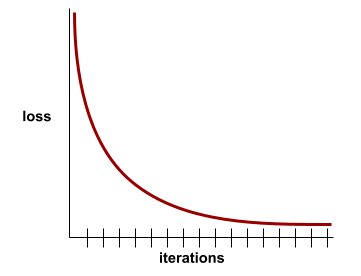Loss curves can plot all of the following types of loss:

## loss function

#fundamentals

During training or testing, a mathematical function that calculates the loss on a batch of examples. A loss function returns a lower loss for models that makes good predictions than for models that make bad predictions.

The goal of training is typically to minimize the loss that a loss function returns.

Many different kinds of loss functions exist. Pick the appropriate loss function for the kind of model you are building. For example:

## loss surface

A graph of weight(s) vs. loss. Gradient descent aims to find the weight(s) for which the loss surface is at a local minimum.

## LSTM

#seq

Abbreviation for Long Short-Term Memory.

## machine learning

#fundamentals

A program or system that trains a model from input data. The trained model can make useful predictions from new (never-before-seen) data drawn from the same distribution as the one used to train the model.

Machine learning also refers to the field of study concerned with these programs or systems.

## majority class

#fundamentals

The more common label in a class-imbalanced dataset. For example, given a dataset containing 99% negative labels and 1% positive labels, the negative labels are the majority class.

Contrast with minority class.

## Markov decision process (MDP)

#rl

A graph representing the decision-making model where decisions (or actions) are taken to navigate a sequence of states under the assumption that the Markov property holds. In reinforcement learning, these transitions between states return a numerical reward.

## Markov property

#rl

A property of certain environments, where state transitions are entirely determined by information implicit in the current state and the agent’s action.

#language

A language model that predicts the probability of candidate tokens to fill in blanks in a sequence. For instance, a masked language model can calculate probabilities for candidate word(s) to replace the underline in the following sentence:

The ____ in the hat came back.

The literature typically uses the string "MASK" instead of an underline. For example:

The "MASK" in the hat came back.

Most modern masked language models are bidirectional.

## matplotlib

An open-source Python 2D plotting library. matplotlib helps you visualize different aspects of machine learning.

## matrix factorization

#recsystems

In math, a mechanism for finding the matrices whose dot product approximates a target matrix.

In recommendation systems, the target matrix often holds users' ratings on items. For example, the target matrix for a movie recommendation system might look something like the following, where the positive integers are user ratings and 0 means that the user didn't rate the movie:

Casablanca The Philadelphia Story Black Panther Wonder Woman Pulp Fiction
User 1 5.0 3.0 0.0 2.0 0.0
User 2 4.0 0.0 0.0 1.0 5.0
User 3 3.0 1.0 4.0 5.0 0.0

The movie recommendation system aims to predict user ratings for unrated movies. For example, will User 1 like Black Panther?

One approach for recommendation systems is to use matrix factorization to generate the following two matrices:

• A user matrix, shaped as the number of users X the number of embedding dimensions.
• An item matrix, shaped as the number of embedding dimensions X the number of items.

For example, using matrix factorization on our three users and five items could yield the following user matrix and item matrix:

User Matrix                 Item Matrix

1.1   2.3           0.9   0.2   1.4    2.0   1.2
0.6   2.0           1.7   1.2   1.2   -0.1   2.1
2.5   0.5


The dot product of the user matrix and item matrix yields a recommendation matrix that contains not only the original user ratings but also predictions for the movies that each user hasn't seen. For example, consider User 1's rating of Casablanca, which was 5.0. The dot product corresponding to that cell in the recommendation matrix should hopefully be around 5.0, and it is:

(1.1 * 0.9) + (2.3 * 1.7) = 4.9


More importantly, will User 1 like Black Panther? Taking the dot product corresponding to the first row and the third column yields a predicted rating of 4.3:

(1.1 * 1.4) + (2.3 * 1.2) = 4.3


Matrix factorization typically yields a user matrix and item matrix that, together, are significantly more compact than the target matrix.

## Mean Absolute Error (MAE)

The average loss per example when L1 loss is used. Calculate Mean Absolute Error as follows:

1. Calculate the L1 loss for a batch.
2. Divide the L1 loss by the number of examples in the batch.

For example, consider the calculation of L1 loss on the following batch of five examples:

Actual value of example Model's predicted value Loss (difference between actual and predicted)
7 6 1
5 4 1
8 11 3
4 6 2
9 8 1
8 = L1 loss

So, L1 loss is 8 and the number of examples is 5. Therefore, the Mean Absolute Error is:

Mean Absolute Error = L1 loss / Number of Examples
Mean Absolute Error = 8/5 = 1.6


Contrast Mean Absolute Error with Mean Squared Error and Root Mean Squared Error.

## Mean Squared Error (MSE)

The average loss per example when L2 loss is used. Calculate Mean Squared Error as follows:

1. Calculate the L2 loss for a batch.
2. Divide the L2 loss by the number of examples in the batch.

For example, consider the loss on the following batch of five examples:

Actual value Model's prediction Loss Squared loss
7 6 1 1
5 4 1 1
8 11 3 9
4 6 2 4
9 8 1 1
16 = L2 loss

Therefore, the Mean Squared Error is:

Mean Squared Error = L2 loss / Number of Examples
Mean Squared Error = 16/5 = 3.2


Mean Squared Error is a popular training optimizer, particularly for linear regression.

Contrast Mean Squared Error with Mean Absolute Error and Root Mean Squared Error.

TensorFlow Playground uses Mean Squared Error to calculate loss values.

## mesh

#TensorFlow

In ML parallel programming, a term associated with assigning the data and model to TPU chips, and defining how these values will be sharded or replicated.

Mesh is an overloaded term that can mean either of the following:

• A physical layout of TPU chips.
• An abstract logical construct for mapping the data and model to the TPU chips.

In either case, a mesh is specified as a shape.

## meta-learning

#language

A subset of machine learning that discovers or improves a learning algorithm. A meta-learning system can also aim to train a model to quickly learn a new task from a small amount of data or from experience gained in previous tasks. Meta-learning algorithms generally try to achieve the following:

• Improve/learn hand-engineered features (such as an initializer or an optimizer).
• Be more data-efficient and compute-efficient.
• Improve generalization.

Meta-learning is related to few-shot learning.

## metric

#TensorFlow

A statistic that you care about.

An objective is a metric that a machine learning system tries to optimize.

## Metrics API (tf.metrics)

A TensorFlow API for evaluating models. For example, tf.metrics.accuracy determines how often a model's predictions match labels.

## mini-batch

#fundamentals

A small, randomly selected subset of a batch processed in one iteration. The batch size of a mini-batch is usually between 10 and 1,000 examples.

For example, suppose the entire training set (the full batch) consists of 1,000 examples. Further suppose that you set the batch size of each mini-batch to 20. Therefore, each iteration determines the loss on a random 20 of the 1,000 examples and then adjusts the weights and biases accordingly.

It is much more efficient to calculate the loss on a mini-batch than the loss on all the examples in the full batch.

A gradient descent algorithm that uses mini-batches. In other words, mini-batch stochastic gradient descent estimates the gradient based on a small subset of the training data. Regular stochastic gradient descent uses a mini-batch of size 1.

## minimax loss

A loss function for generative adversarial networks, based on the cross-entropy between the distribution of generated data and real data.

Minimax loss is used in the first paper to describe generative adversarial networks.

## minority class

#fundamentals

The less common label in a class-imbalanced dataset. For example, given a dataset containing 99% negative labels and 1% positive labels, the positive labels are the minority class.

Contrast with majority class.

## ML

Abbreviation for machine learning.

## MNIST

#image

A public-domain dataset compiled by LeCun, Cortes, and Burges containing 60,000 images, each image showing how a human manually wrote a particular digit from 0–9. Each image is stored as a 28x28 array of integers, where each integer is a grayscale value between 0 and 255, inclusive.

MNIST is a canonical dataset for machine learning, often used to test new machine learning approaches. For details, see The MNIST Database of Handwritten Digits.

## modality

#language

A high-level data category. For example, numbers, text, images, video, and audio are five different modalities.

## model

#fundamentals

In general, any mathematical construct that processes input data and returns output. Phrased differently, a model is the set of parameters and structure needed for a system to make predictions. In supervised machine learning, a model takes an example as input and infers a prediction as output. Within supervised machine learning, models differ somewhat. For example:

• A linear regression model consists of a set of weights and a bias.
• A neural network model consists of:
• A set of hidden layers, each containing one or more neurons.
• The weights and bias associated with each neuron.
• A decision tree model consists of:
• The shape of the tree; that is, the pattern in which the conditions and leaves are connected.
• The conditions and leaves.

You can save, restore, or make copies of a model.

Unsupervised machine learning also generates models, typically a function that can map an input example to the most appropriate cluster.

## model capacity

The complexity of problems that a model can learn. The more complex the problems that a model can learn, the higher the model’s capacity. A model’s capacity typically increases with the number of model parameters. For a formal definition of classifier capacity, see VC dimension.

## model parallelism

#language

A way of scaling training or inference that puts different parts of one model on different devices. Model parallelism enables models that are too big to fit on a single device.

To implement model parallelism, a system typically does the following:

1. Shards (divides) the model into smaller parts.
2. Distributes the training of those smaller parts across multiple processors. Each processor trains its own part of the model.
3. Combines the results to create a single model.

Model parallelism slows training.

## model training

The process of determining the best model.

## Momentum

A sophisticated gradient descent algorithm in which a learning step depends not only on the derivative in the current step, but also on the derivatives of the step(s) that immediately preceded it. Momentum involves computing an exponentially weighted moving average of the gradients over time, analogous to momentum in physics. Momentum sometimes prevents learning from getting stuck in local minima.

## multi-class classification

#fundamentals

In supervised learning, a classification problem in which the dataset contains more than two classes of labels. For example, the labels in the Iris dataset must be one of the following three classes:

• Iris setosa
• Iris virginica
• Iris versicolor

A model trained on the Iris dataset that predicts Iris type on new examples is performing multi-class classification.

In contrast, classification problems that distinguish between exactly two classes are binary classification models. For example, an email model that predicts either spam or not spam is a binary classification model.

In clustering problems, multi-class classification refers to more than two clusters.

## multi-class logistic regression

Using logistic regression in multi-class classification problems.

#language

An extension of self-attention that applies the self-attention mechanism multiple times for each position in the input sequence.

## multimodal model

#language

A model whose inputs and/or outputs include more than one modality. For example, consider a model that takes both an image and a text caption (two modalities) as features, and outputs a score indicating how appropriate the text caption is for the image. So, this model's inputs are multimodal and the output is unimodal.

## multinomial classification

Synonym for multi-class classification.

## multinomial regression

Synonym for multi-class logistic regression.

A machine learning technique in which a single model is trained to perform multiple tasks.

Multitask models are created by training on data that is appropriate for each of the different tasks. This allows the model to learn to share information across the tasks, which helps the model learn more effectively.

A model trained for multiple tasks often has improved generalization abilities and can be more robust at handling different types of data.

## NaN trap

When one number in your model becomes a NaN during training, which causes many or all other numbers in your model to eventually become a NaN.

NaN is an abbreviation for Not a Number.

## natural language understanding

#language

Determining a user's intentions based on what the user typed or said. For example, a search engine uses natural language understanding to determine what the user is searching for based on what the user typed or said.

## negative class

#fundamentals

In binary classification, one class is termed positive and the other is termed negative. The positive class is the thing or event that the model is testing for and the negative class is the other possibility. For example:

• The negative class in a medical test might be "not tumor."
• The negative class in an email classifier might be "not spam."

Contrast with positive class.

## negative sampling

Synonym for candidate sampling.

## Neural Architecture Search (NAS)

A technique for automatically designing the architecture of a neural network. NAS algorithms can reduce the amount of time and resources required to train a neural network.

NAS typically uses:

• A search space, which is a set of possible architectures.
• A fitness function, which is a measure of how well a particular architecture performs on a given task.

NAS algorithms often start with a small set of possible architectures and gradually expand the search space as the algorithm learns more about what architectures are effective. The fitness function is typically based on the performance of the architecture on a training set, and the algorithm is typically trained using a reinforcement learning technique.

NAS algorithms have proven effective in finding high-performing architectures for a variety of tasks, including image classification, text classification, and machine translation.

## neural network

#fundamentals

A model containing at least one hidden layer. A deep neural network is a type of neural network containing more than one hidden layer. For example, the following diagram shows a deep neural network containing two hidden layers.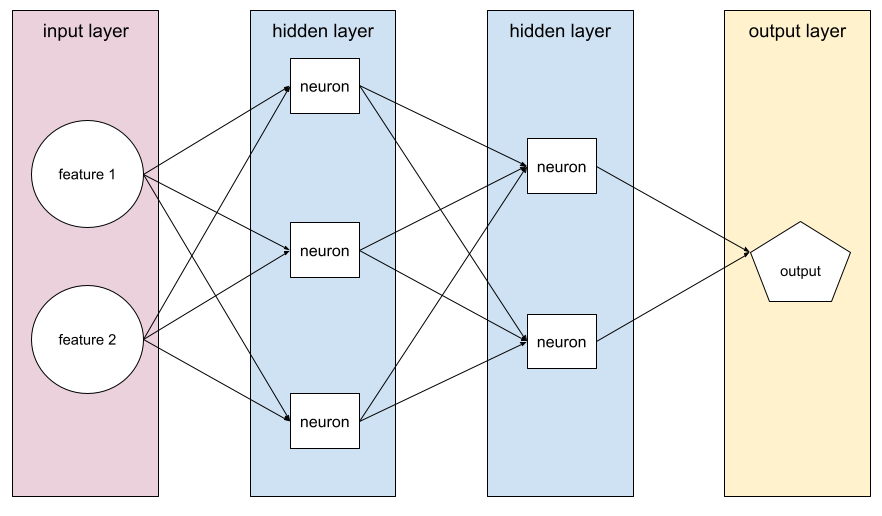Each neuron in a neural network connects to all of the nodes in the next layer. For example, in the preceding diagram, notice that each of the three neurons in the first hidden layer separately connect to both of the two neurons in the second hidden layer.

Neural networks implemented on computers are sometimes called artificial neural networks to differentiate them from neural networks found in brains and other nervous systems.

Some neural networks can mimic extremely complex nonlinear relationships between different features and the label.

## neuron

#fundamentals

In machine learning, a distinct unit within a hidden layer of a neural network. Each neuron performs the following two-step action:

1. Calculates the weighted sum of input values multiplied by their corresponding weights.
2. Passes the weighted sum as input to an activation function.

A neuron in the first hidden layer accepts inputs from the feature values in the input layer. A neuron in any hidden layer beyond the first accepts inputs from the neurons in the preceding hidden layer. For example, a neuron in the second hidden layer accepts inputs from the neurons in the first hidden layer.

The following illustration highlights two neurons and their inputs.A neuron in a neural network mimics the behavior of neurons in brains and other parts of nervous systems.

## N-gram

#seq
#language

An ordered sequence of N words. For example, truly madly is a 2-gram. Because order is relevant, madly truly is a different 2-gram than truly madly.

N Name(s) for this kind of N-gram Examples
2 bigram or 2-gram to go, go to, eat lunch, eat dinner
3 trigram or 3-gram ate too much, three blind mice, the bell tolls
4 4-gram walk in the park, dust in the wind, the boy ate lentils

Many natural language understanding models rely on N-grams to predict the next word that the user will type or say. For example, suppose a user typed three blind. An NLU model based on trigrams would likely predict that the user will next type mice.

Contrast N-grams with bag of words, which are unordered sets of words.

## NLU

#language

Abbreviation for natural language understanding.

## node (decision tree)

#df

In a decision tree, any condition or leaf.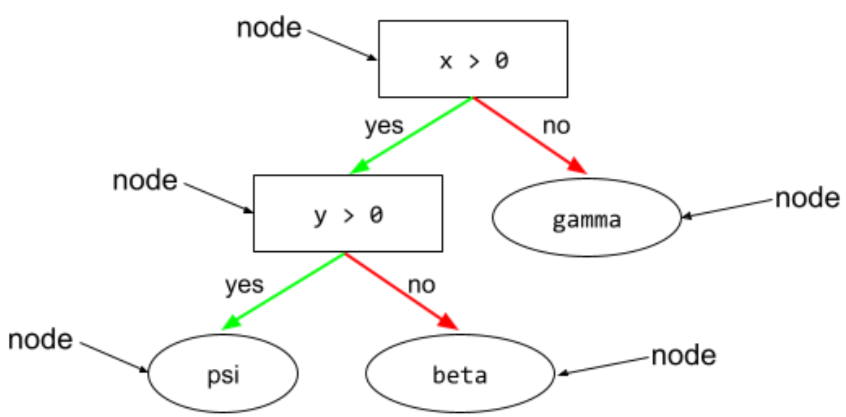## node (neural network)

#fundamentals

A neuron in a hidden layer.

## node (TensorFlow graph)

#TensorFlow

An operation in a TensorFlow graph.

## noise

Broadly speaking, anything that obscures the signal in a dataset. Noise can be introduced into data in a variety of ways. For example:

• Human raters make mistakes in labeling.
• Humans and instruments mis-record or omit feature values.

## non-binary condition

#df

A condition containing more than two possible outcomes. For example, the following non-binary condition contains three possible outcomes: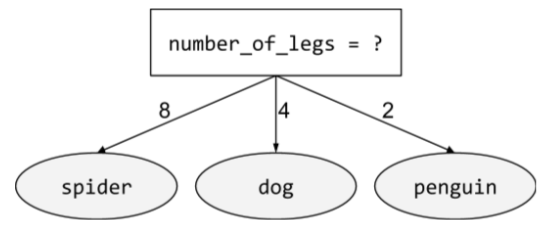## nonlinear

#fundamentals

A relationship between two or more variables that can't be represented solely through addition and multiplication. A linear relationship can be represented as a line; a nonlinear relationship can't be represented as a line. For example, consider two models that each relate a single feature to a single label. The model on the left is linear and the model on the right is nonlinear: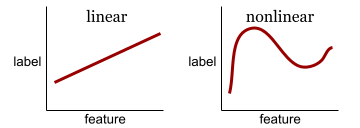## non-response bias

#fairness

See selection bias.

## nonstationarity

#fundamentals

A feature whose values change across one or more dimensions, usually time. For example, consider the following examples of nonstationarity:

• The number of swimsuits sold at a particular store varies with the season.
• The quantity of a particular fruit harvested in a particular region is zero for much of the year but large for a brief period.
• Due to climate change, annual mean temperatures are shifting.

Contrast with stationarity.

## normalization

#fundamentals

Broadly speaking, the process of converting a variable's actual range of values into a standard range of values, such as:

• -1 to +1
• 0 to 1
• the normal distribution

For example, suppose the actual range of values of a certain feature is 800 to 2,400. As part of feature engineering, you could normalize the actual values down to a standard range, such as -1 to +1.

Normalization is a common task in feature engineering. Models usually train faster (and produce better predictions) when every numerical feature in the feature vector has roughly the same range.

## novelty detection

The process of determining whether a new (novel) example comes from the same distribution as the training set. In other words, after training on the training set, novelty detection determines whether a new example (during inference or during additional training) is an outlier.

Contrast with outlier detection.

## numerical data

#fundamentals

Features represented as integers or real-valued numbers. For example, a house valuation model would probably represent the size of a house (in square feet or square meters) as numerical data. Representing a feature as numerical data indicates that the feature's values have a mathematical relationship to the label. That is, the number of square meters in a house probably has some mathematical relationship to the value of the house.

Not all integer data should be represented as numerical data. For example, postal codes in some parts of the world are integers; however, integer postal codes should not be represented as numerical data in models. That's because a postal code of 20000 is not twice (or half) as potent as a postal code of 10000. Furthermore, although different postal codes do correlate to different real estate values, we can't assume that real estate values at postal code 20000 are twice as valuable as real estate values at postal code 10000. Postal codes should be represented as categorical data instead.

Numerical features are sometimes called continuous features.

## NumPy

An open-source math library that provides efficient array operations in Python. pandas is built on NumPy.

## objective

A metric that your algorithm is trying to optimize.

## objective function

The mathematical formula or metric that a model aims to optimize. For example, the objective function for linear regression is usually Mean Squared Loss. Therefore, when training a linear regression model, training aims to minimize Mean Squared Loss.

In some cases, the goal is to maximize the objective function. For example, if the objective function is accuracy, the goal is to maximize accuracy.

## oblique condition

#df

In a decision tree, a condition that involves more than one feature. For example, if height and width are both features, then the following is an oblique condition:

  height > width


Contrast with axis-aligned condition.

## offline

#fundamentals

Synonym for static.

## offline inference

#fundamentals

The process of a model generating a batch of predictions and then caching (saving) those predictions. Apps can then access the desired prediction from the cache rather than rerunning the model.

For example, consider a model that generates local weather forecasts (predictions) once every four hours. After each model run, the system caches all the local weather forecasts. Weather apps retrieve the forecasts from the cache.

Offline inference is also called static inference.

Contrast with online inference.

## one-hot encoding

#fundamentals

Representing categorical data as a vector in which:

• One element is set to 1.
• All other elements are set to 0.

One-hot encoding is commonly used to represent strings or identifiers that have a finite set of possible values. For example, suppose a certain categorical feature named Scandinavia has five possible values:

• "Denmark"
• "Sweden"
• "Norway"
• "Finland"
• "Iceland"

One-hot encoding could represent each of the five values as follows:

country Vector
"Denmark" 1 0 0 0 0
"Sweden" 0 1 0 0 0
"Norway" 0 0 1 0 0
"Finland" 0 0 0 1 0
"Iceland" 0 0 0 0 1

Thanks to one-hot encoding, a model can learn different connections based on each of the five countries.

Representing a feature as numerical data is an alternative to one-hot encoding. Unfortunately, representing the Scandinavian countries numerically is not a good choice. For example, consider the following numeric representation:

• "Denmark" is 0
• "Sweden" is 1
• "Norway" is 2
• "Finland" is 3
• "Iceland" is 4

With numeric encoding, a model would interpret the raw numbers mathematically and would try to train on those numbers. However, Iceland isn't actually twice as much (or half as much) of something as Norway, so the model would come to some strange conclusions.

## one-shot learning

A machine learning approach, often used for object classification, designed to learn effective classifiers from a single training example.

## one-shot prompting

#language
#generativeAI

A prompt that contains one example demonstrating how the large language model should respond. For example, the following prompt contains one example showing a large language model how it should answer a query.

Parts of one prompt Notes
What is the official currency of the specified country? The question you want the LLM to answer.
France: EUR One example.
India: The actual query.

Compare and contrast one-shot prompting with the following terms:

## one-vs.-all

#fundamentals

Given a classification problem with N classes, a solution consisting of N separate binary classifiers—one binary classifier for each possible outcome. For example, given a model that classifies examples as animal, vegetable, or mineral, a one-vs.-all solution would provide the following three separate binary classifiers:

• animal vs. not animal
• vegetable vs. not vegetable
• mineral vs. not mineral

## online

#fundamentals

Synonym for dynamic.

## online inference

#fundamentals

Generating predictions on demand. For example, suppose an app passes input to a model and issues a request for a prediction. A system using online inference responds to the request by running the model (and returning the prediction to the app).

Contrast with offline inference.

## operation (op)

#TensorFlow

In TensorFlow, any procedure that creates, manipulates, or destroys a Tensor. For example, a matrix multiply is an operation that takes two Tensors as input and generates one Tensor as output.

## Optax

A gradient processing and optimization library for JAX. Optax facilitates research by providing building blocks that can be recombined in custom ways to optimize parametric models such as deep neural networks. Other goals include:

• Providing readable, well-tested, efficient implementations of core components.
• Improving productivity by making it possible to combine low level ingredients into custom optimizers (or other gradient processing components).
• Accelerating adoption of new ideas by making it easy for anyone to contribute.

## optimizer

A specific implementation of the gradient descent algorithm. Popular optimizers include:

## out-group homogeneity bias

#fairness

The tendency to see out-group members as more alike than in-group members when comparing attitudes, values, personality traits, and other characteristics. In-group refers to people you interact with regularly; out-group refers to people you do not interact with regularly. If you create a dataset by asking people to provide attributes about out-groups, those attributes may be less nuanced and more stereotyped than attributes that participants list for people in their in-group.

For example, Lilliputians might describe the houses of other Lilliputians in great detail, citing small differences in architectural styles, windows, doors, and sizes. However, the same Lilliputians might simply declare that Brobdingnagians all live in identical houses.

Out-group homogeneity bias is a form of group attribution bias.

## outlier detection

The process of identifying outliers in a training set.

Contrast with novelty detection.

## outliers

Values distant from most other values. In machine learning, any of the following are outliers:

• Input data whose values are more than roughly 3 standard deviations from the mean.
• Weights with high absolute values.
• Predicted values relatively far away from the actual values.

For example, suppose that widget-price is a feature of a certain model. Assume that the mean widget-price is 7 Euros with a standard deviation of 1 Euro. Examples containing a widget-price of 12 Euros or 2 Euros would therefore be considered outliers because each of those prices is five standard deviations from the mean.

Outliers are often caused by typos or other input mistakes. In other cases, outliers aren't mistakes; after all, values five standard deviations away from the mean are rare but hardly impossible.

Outliers often cause problems in model training. Clipping is one way of managing outliers.

## out-of-bag evaluation (OOB evaluation)

#df

A mechanism for evaluating the quality of a decision forest by testing each decision tree against the examples not used during training of that decision tree. For example, in the following diagram, notice that the system trains each decision tree on about two-thirds of the examples and then evaluates against the remaining one-third of the examples.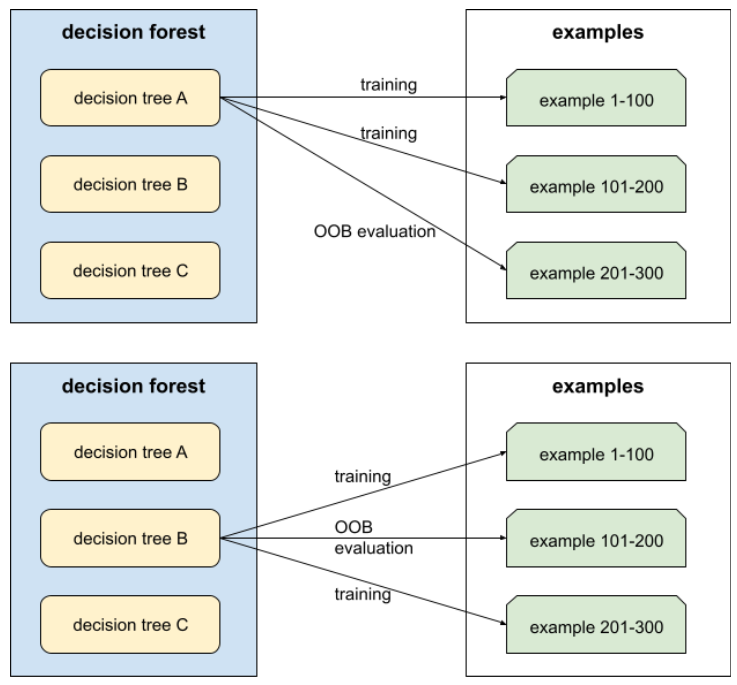Out-of-bag evaluation is a computationally efficient and conservative approximation of the cross-validation mechanism. In cross-validation, one model is trained for each cross-validation round (for example, 10 models are trained in a 10-fold cross-validation). With OOB evaluation, a single model is trained. Because bagging withholds some data from each tree during training, OOB evaluation can use that data to approximate cross-validation.

## output layer

#fundamentals

The "final" layer of a neural network. The output layer contains the prediction.

The following illustration shows a small deep neural network with an input layer, two hidden layers, and an output layer: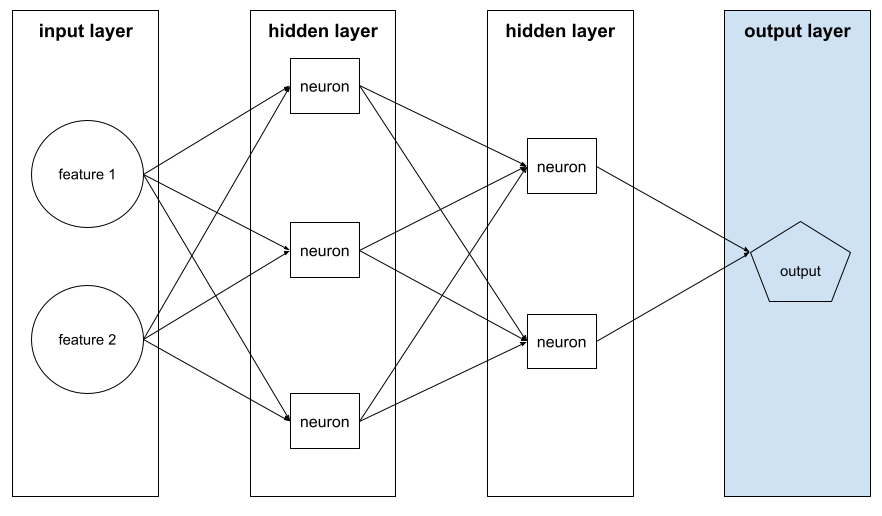## overfitting

#fundamentals

Creating a model that matches the training data so closely that the model fails to make correct predictions on new data.

Regularization can reduce overfitting. Training on a large and diverse training set can also reduce overfitting.

## oversampling

Reusing the examples of a minority class in a class-imbalanced dataset in order to create a more balanced training set.

For example, consider a binary classification problem in which the ratio of the majority class to the minority class is 5,000:1. If the dataset contains a million examples, then the dataset contains only about 200 examples of the minority class, which might be too few examples for effective training. To overcome this deficiency, you might oversample (reuse) those 200 examples multiple times, possibly yielding sufficient examples for useful training.

You need to be careful about over overfitting when oversampling.

Contrast with undersampling.

## packed data

An approach for storing data more efficiently.

Packed data stores data either by using a compressed format or in some other way that allows it to be accessed more efficiently. Packed data minimizes the amount of memory and computation required to access it, leading to faster training and more efficient model inference.

Packed data is often used with other techniques, such as data augmentation and regularization, further improving the performance of models.

## pandas

#fundamentals

A column-oriented data analysis API built on top of numpy. Many machine learning frameworks, including TensorFlow, support pandas data structures as inputs. See the pandas documentation for details.

## parameter

#fundamentals

The weights and biases that a model learns during training. For example, in a linear regression model, the parameters consist of the bias (b) and all the weights (w1, w2, and so on) in the following formula:

$$y' = b + w_1x_1 + w_2x_2 + … w_nx_n$$

In contrast, hyperparameter are the values that you (or a hyperparameter turning service) supply to the model. For example, learning rate is a hyperparameter.

## parameter-efficient tuning

#language
#generativeAI

A set of techniques to fine-tune a large pre-trained language model (PLM) more efficiently than full fine-tuning. Parameter-efficient tuning typically fine-tunes far fewer parameters than full fine-tuning, yet generally produces a large language model that performs as well (or almost as well) as a large language model built from full fine-tuning.

Compare and contrast parameter-efficient tuning with:

Parameter-efficient tuning is also known as parameter-efficient fine-tuning.

## Parameter Server (PS)

#TensorFlow

A job that keeps track of a model's parameters in a distributed setting.

## parameter update

The operation of adjusting a model's parameters during training, typically within a single iteration of gradient descent.

## partial derivative

A derivative in which all but one of the variables is considered a constant. For example, the partial derivative of f(x, y) with respect to x is the derivative of f considered as a function of x alone (that is, keeping y constant). The partial derivative of f with respect to x focuses only on how x is changing and ignores all other variables in the equation.

## participation bias

#fairness

Synonym for non-response bias. See selection bias.

## partitioning strategy

The algorithm by which variables are divided across parameter servers.

## Pax

A programming framework designed for training large-scale neural network models so large that they span multiple TPU accelerator chip slices or pods.

Pax is built on Flax, which is built on JAX.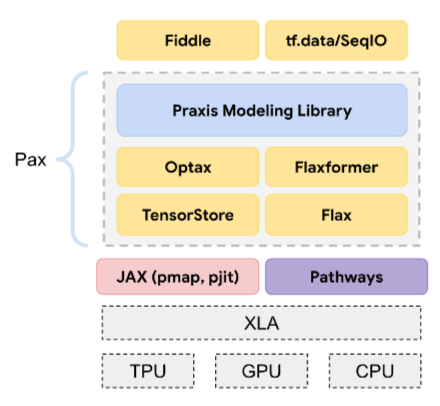## perceptron

A system (either hardware or software) that takes in one or more input values, runs a function on the weighted sum of the inputs, and computes a single output value. In machine learning, the function is typically nonlinear, such as ReLU, sigmoid, or tanh. For example, the following perceptron relies on the sigmoid function to process three input values:

$$f(x_1, x_2, x_3) = \text{sigmoid}(w_1 x_1 + w_2 x_2 + w_3 x_3)$$

In the following illustration, the perceptron takes three inputs, each of which is itself modified by a weight before entering the perceptron:Perceptrons are the neurons in neural networks.

## performance

Overloaded term with the following meanings:

• The traditional meaning within software engineering. Namely: How fast (or efficiently) does this piece of software run?
• The meaning within machine learning. Here, performance answers the following question: How correct is this model? That is, how good are the model's predictions?

## permutation variable importances

#df

A type of variable importance that evaluates the increase in the prediction error of a model after permuting the feature’s values. Permutation variable importance is a model agnostic metric.

## perplexity

One measure of how well a model is accomplishing its task. For example, suppose your task is to read the first few letters of a word a user is typing on a smartphone keyboard, and to offer a list of possible completion words. Perplexity, P, for this task is approximately the number of guesses you need to offer in order for your list to contain the actual word the user is trying to type.

Perplexity is related to cross-entropy as follows:

$$P= 2^{-\text{cross entropy}}$$

## pipeline

The infrastructure surrounding a machine learning algorithm. A pipeline includes gathering the data, putting the data into training data files, training one or more models, and exporting the models to production.

## pipelining

#language

A form of model parallelism in which a model's processing is divided into consecutive stages and each stage is executed on a different device. While a stage is processing one batch, the preceding stage can work on the next batch.

## pjit

A JAX function that splits code to run across multiple accelerator chips. The user passes a function to pjit, which returns a function that has the equivalent semantics but is compiled into an XLA computation that runs across multiple devices (such as GPUs or TPU cores).

pjit enables users to shard computations without rewriting them by using the SPMD partitioner.

As of March 2023, pjit has been merged with jit. Refer to Distributed arrays and automatic parallelization for more details.

## PLM

#language
#generativeAI

Abbreviation for pre-trained language model.

## pmap

A JAX function that executes copies of an input function on multiple underlying hardware devices (CPUs, GPUs, or TPUs), with different input values. pmap relies on SPMD.

## policy

#rl

In reinforcement learning, an agent's probabilistic mapping from states to actions.

## pooling

#image

Reducing a matrix (or matrices) created by an earlier convolutional layer to a smaller matrix. Pooling usually involves taking either the maximum or average value across the pooled area. For example, suppose we have the following 3x3 matrix:A pooling operation, just like a convolutional operation, divides that matrix into slices and then slides that convolutional operation by strides. For example, suppose the pooling operation divides the convolutional matrix into 2x2 slices with a 1x1 stride. As the following diagram illustrates, four pooling operations take place. Imagine that each pooling operation picks the maximum value of the four in that slice:Pooling helps enforce translational invariance in the input matrix.

Pooling for vision applications is known more formally as spatial pooling. Time-series applications usually refer to pooling as temporal pooling. Less formally, pooling is often called subsampling or downsampling.

## positional encoding

#language

A technique to add information about the position of a token in a sequence to the token's embedding. Transformer models use positional encoding to better understand the relationship between different parts of the sequence.

A common implementation of positional encoding uses a sinusoidal function. (Specifically, the frequency and amplitude of the sinusoidal function are determined by the position of the token in the sequence.) This technique enables a Transformer model to learn to attend to different parts of the sequence based on their position.

## positive class

#fundamentals

The class you are testing for.

For example, the positive class in a cancer model might be "tumor." The positive class in an email classifier might be "spam."

Contrast with negative class.

## post-processing

#fairness
#fundamentals

Adjusting the output of a model after the model has been run. Post-processing can be used to enforce fairness constraints without modifying models themselves.

For example, one might apply post-processing to a binary classifier by setting a classification threshold such that equality of opportunity is maintained for some attribute by checking that the true positive rate is the same for all values of that attribute.

## PR AUC (area under the PR curve)

Area under the interpolated precision-recall curve, obtained by plotting (recall, precision) points for different values of the classification threshold. Depending on how it's calculated, PR AUC may be equivalent to the average precision of the model.

## Praxis

A core, high-performance ML library of Pax. Praxis is often called the "Layer library".

Praxis contains not just the definitions for the Layer class, but most of its supporting components as well, including:

Praxis provides the definitions for the Model class.

## precision

A metric for classification models that answers the following question:

When the model predicted the positive class, what percentage of the predictions were correct?

Here is the formula:

$$\text{Precision} = \frac{\text{true positives}} {\text{true positives} + \text{false positives}}$$

where:

• true positive means the model correctly predicted the positive class.
• false positive means the model mistakenly predicted the positive class.

For example, suppose a model made 200 positive predictions. Of these 200 positive predictions:

• 150 were true positives.
• 50 were false positives.

In this case:

$$\text{Precision} = \frac{\text{150}} {\text{150} + \text{50}} = 0.75$$

Contrast with accuracy and recall.

## precision-recall curve

A curve of precision vs. recall at different classification thresholds.

## prediction

#fundamentals

A model's output. For example:

• The prediction of a binary classification model is either the positive class or the negative class.
• The prediction of a multi-class classification model is one class.
• The prediction of a linear regression model is a number.

## prediction bias

A value indicating how far apart the average of predictions is from the average of labels in the dataset.

Not to be confused with the bias term in machine learning models or with bias in ethics and fairness.

## predictive ML

Any traditional ("classic") machine learning system.

The term predictive ML doesn't have a formal definition. Rather, the term distinguishes a category of ML systems not based on generative AI.

## predictive parity

#fairness

A fairness metric that checks whether, for a given classifier, the precision rates are equivalent for subgroups under consideration.

For example, a model that predicts college acceptance would satisfy predictive parity for nationality if its precision rate is the same for Lilliputians and Brobdingnagians.

Predictive parity is sometime also called predictive rate parity.

See "Fairness Definitions Explained" (section 3.2.1) for a more detailed discussion of predictive parity.

## predictive rate parity

#fairness

Another name for predictive parity.

## preprocessing

#fairness
Processing data before it's used to train a model. Preprocessing could be as simple as removing words from an English text corpus that don't occur in the English dictionary, or could be as complex as re-expressing data points in a way that eliminates as many attributes that are correlated with sensitive attributes as possible. Preprocessing can help satisfy fairness constraints.

## pre-trained model

#language
#image
#generativeAI

Models or model components (such as an embedding vector) that have been already been trained. Sometimes, you'll feed pre-trained embedding vectors into a neural network. Other times, your model will train the embedding vectors themselves rather than rely on the pre-trained embeddings.

The term pre-trained language model refers to a large language model that has gone through pre-training.

## pre-training

#language
#image
#generativeAI

The initial training of a model on a large dataset. Some pre-trained models are clumsy giants and must typically be refined through additional training. For example, ML experts might pre-train a large language model on a vast text dataset, such as all the English pages in Wikipedia. Following pre-training, the resulting model might be further refined through any of the following techniques:

## prior belief

What you believe about the data before you begin training on it. For example, L2 regularization relies on a prior belief that weights should be small and normally distributed around zero.

## probabilistic regression model

A regression model that uses not only the weights for each feature, but also the uncertainty of those weights. A probabilistic regression model generates a prediction and the uncertainty of that prediction. For example, a probabilistic regression model might yield a prediction of 325 with a standard deviation of 12. For more information about probabilistic regression models, see this Colab on tensorflow.org.

## prompt

#language
#generativeAI

Any text entered as input to a large language model to condition the model to behave in a certain way. Prompts can be as short as a phrase or arbitrarily long (for example, the entire text of a novel). Prompts fall into multiple categories, including those shown in the following table:

Prompt category Example Notes
Question How fast can a pigeon fly?
Instruction Write a funny poem about arbitrage. A prompt that asks the large language model to do something.
Example Translate Markdown code to HTML. For example:
Markdown: * list item
HTML: <ul> <li>list item</li> </ul>
The first sentence in this example prompt is an instruction. The remainder of the prompt is the example.
Role Explain why gradient descent is used in machine learning training to a PhD in Physics. The first part of the sentence is an instruction; the phrase "to a PhD in Physics" is the role portion.
Partial input for the model to complete The Prime Minister of the United Kingdom lives at A partial input prompt can either end abruptly (as this example does) or end with an underscore.

A generative AI model can respond to a prompt with text, code, images, embeddings, videos…almost anything.

## prompt-based learning

#language
#generativeAI

A capability of certain models that enables them to adapt their behavior in response to arbitrary text input (prompts). In a typical prompt-based learning paradigm, a large language model responds to a prompt by generating text. For example, suppose a user enters the following prompt:

Summarize Newton's Third Law of Motion.

A model capable of prompt-based learning isn't specifically trained to answer the previous prompt. Rather, the model "knows" a lot of facts about physics, a lot about general language rules, and a lot about what constitutes generally useful answers. That knowledge is sufficient to provide a (hopefully) useful answer. Additional human feedback ("That answer was too complicated." or "What's a reaction?") enables some prompt-based learning systems to gradually improve the usefulness of their answers.

## prompt design

#language
#generativeAI

Synonym for prompt engineering.

## prompt engineering

#language
#generativeAI

The art of creating prompts that elicit the desired responses from a large language model. Humans perform prompt engineering. Writing well-structured prompts is an essential part of ensuring useful responses from a large language model. Prompt engineering depends on many factors, including:

• The dataset used to pre-train and possibly fine-tune the large language model.
• The temperature and other decoding parameters that the model uses to generate responses.

See Introduction to prompt design for more details on writing helpful prompts.

Prompt design is a synonym for prompt engineering.

## prompt tuning

#language
#generativeAI

A parameter efficient tuning mechanism that learns a "prefix" that the system prepends to the actual prompt.

One variation of prompt tuning—sometimes called prefix tuning—is to prepend the prefix at every layer. In contrast, most prompt tuning only adds a prefix to the input layer.

## proxy labels

#fundamentals

Data used to approximate labels not directly available in a dataset.

For example, suppose you must train a model to predict employee stress level. Your dataset contains a lot of predictive features but doesn't contain a label named stress level. Undaunted, you pick "workplace accidents" as a proxy label for stress level. After all, employees under high stress get into more accidents than calm employees. Or do they? Maybe workplace accidents actually rise and fall for multiple reasons.

As a second example, suppose you want is it raining? to be a Boolean label for your dataset, but your dataset doesn't contain rain data. If photographs are available, you might establish pictures of people carrying umbrellas as a proxy label for is it raining? Is that a good proxy label? Possibly, but people in some cultures may be more likely to carry umbrellas to protect against sun than the rain.

Proxy labels are often imperfect. When possible, choose actual labels over proxy labels. That said, when an actual label is absent, pick the proxy label very carefully, choosing the least horrible proxy label candidate.

## proxy (sensitive attributes)

#fairness
An attribute used as a stand-in for a sensitive attribute. For example, an individual's postal code might be used as a proxy for their income, race, or ethnicity.

## pure function

A function whose outputs are based only on its inputs, and that has no side effects. Specifically, a pure function doesn't use or change any global state, such as the contents of a file or the value of a variable outside the function.

Pure functions can be used to create thread-safe code, which is beneficial when sharding model code across multiple accelerator chips.

JAX's function transformation methods require that the input functions are pure functions.

## Q-function

#rl

In reinforcement learning, the function that predicts the expected return from taking an action in a state and then following a given policy.

Q-function is also known as state-action value function.

## Q-learning

#rl

In reinforcement learning, an algorithm that allows an agent to learn the optimal Q-function of a Markov decision process by applying the Bellman equation. The Markov decision process models an environment.

## quantile

Each bucket in quantile bucketing.

## quantile bucketing

Distributing a feature's values into buckets so that each bucket contains the same (or almost the same) number of examples. For example, the following figure divides 44 points into 4 buckets, each of which contains 11 points. In order for each bucket in the figure to contain the same number of points, some buckets span a different width of x-values.## quantization

Overloaded term that could be used in either of two ways:

• Implementing quantile bucketing on a particular feature.
• Transforming data into zeroes and ones for quicker storing, training, and inferring. As Boolean data is more robust to noise and errors than other formats, quantization can improve model correctness. Quantization techniques include rounding, truncating, and binning.

## queue

#TensorFlow

A TensorFlow Operation that implements a queue data structure. Typically used in I/O.

## random forest

#df

An ensemble of decision trees in which each decision tree is trained with a specific random noise, such as bagging.

Random forests are a type of decision forest.

## random policy

#rl

In reinforcement learning, a policy that chooses an action at random.

## ranking

A type of supervised learning whose objective is to order a list of items.

## rank (ordinality)

The ordinal position of a class in a machine learning problem that categorizes classes from highest to lowest. For example, a behavior ranking system could rank a dog's rewards from highest (a steak) to lowest (wilted kale).

## rank (Tensor)

#TensorFlow

The number of dimensions in a Tensor. For instance, a scalar has rank 0, a vector has rank 1, and a matrix has rank 2.

Not to be confused with rank (ordinality).

## rater

#fundamentals

A human who provides labels for examples. "Annotator" is another name for rater.

## recall

A metric for classification models that answers the following question:

When ground truth was the positive class, what percentage of predictions did the model correctly identify as the positive class?

Here is the formula:

$\text{Recall} = \frac{\text{true positives}} {\text{true positives} + \text{false negatives}}$

where:

• true positive means the model correctly predicted the positive class.
• false negative means that the model mistakenly predicted the negative class.

For instance, suppose your model made 200 predictions on examples for which ground truth was the positive class. Of these 200 predictions:

• 180 were true positives.
• 20 were false negatives.

In this case:

$\text{Recall} = \frac{\text{180}} {\text{180} + \text{20}} = 0.9$

## recommendation system

#recsystems

A system that selects for each user a relatively small set of desirable items from a large corpus. For example, a video recommendation system might recommend two videos from a corpus of 100,000 videos, selecting Casablanca and The Philadelphia Story for one user, and Wonder Woman and Black Panther for another. A video recommendation system might base its recommendations on factors such as:

• Movies that similar users have rated or watched.
• Genre, directors, actors, target demographic...

## Rectified Linear Unit (ReLU)

#fundamentals

An activation function with the following behavior:

• If input is negative or zero, then the output is 0.
• If input is positive, then the output is equal to the input.

For example:

• If the input is -3, then the output is 0.
• If the input is +3, then the output is 3.0.

Here is a plot of ReLU: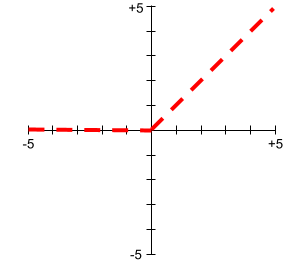ReLU is a very popular activation function. Despite its simple behavior, ReLU still enables a neural network to learn nonlinear relationships between features and the label.

## recurrent neural network

#seq

A neural network that is intentionally run multiple times, where parts of each run feed into the next run. Specifically, hidden layers from the previous run provide part of the input to the same hidden layer in the next run. Recurrent neural networks are particularly useful for evaluating sequences, so that the hidden layers can learn from previous runs of the neural network on earlier parts of the sequence.

For example, the following figure shows a recurrent neural network that runs four times. Notice that the values learned in the hidden layers from the first run become part of the input to the same hidden layers in the second run. Similarly, the values learned in the hidden layer on the second run become part of the input to the same hidden layer in the third run. In this way, the recurrent neural network gradually trains and predicts the meaning of the entire sequence rather than just the meaning of individual words.## regression model

#fundamentals

Informally, a model that generates a numerical prediction. (In contrast, a classification model generates a class prediction.) For example, the following are all regression models:

• A model that predicts a certain house's value, such as 423,000 Euros.
• A model that predicts a certain tree's life expectancy, such as 23.2 years.
• A model that predicts the amount of rain that will fall in a certain city over the next six hours, such as 0.18 inches.

Two common types of regression models are:

• Linear regression, which finds the line that best fits label values to features.
• Logistic regression, which generates a probability between 0.0 and 1.0 that a system typically then maps to a class prediction.

Not every model that outputs numerical predictions is a regression model. In some cases, a numeric prediction is really just a classification model that happens to have numeric class names. For example, a model that predicts a numeric postal code is a classification model, not a regression model.

## regularization

#fundamentals

Any mechanism that reduces overfitting. Popular types of regularization include:

Regularization can also be defined as the penalty on a model's complexity.

## regularization rate

#fundamentals

A number that specifies the relative importance of regularization during training. Raising the regularization rate reduces overfitting but may reduce the model's predictive power. Conversely, reducing or omitting the regularization rate increases overfitting.

## reinforcement learning (RL)

#rl

A family of algorithms that learn an optimal policy, whose goal is to maximize return when interacting with an environment. For example, the ultimate reward of most games is victory. Reinforcement learning systems can become expert at playing complex games by evaluating sequences of previous game moves that ultimately led to wins and sequences that ultimately led to losses.

## Reinforcement Learning from Human Feedback (RLHF)

#generativeAI
#rl

Using feedback from human raters to improve the quality of a model's responses. For example, an RLHF mechanism can ask users to rate the quality of a model's response with a 👍 or 👎 emoji. The system can then adjust its future responses based on that feedback.

## ReLU

#fundamentals

Abbreviation for Rectified Linear Unit.

## replay buffer

#rl

In DQN-like algorithms, the memory used by the agent to store state transitions for use in experience replay.

## replica

A copy of the training set or model, typically on another machine. For example, a system could use the following strategy for implementing data parallelism:

1. Place replicas of an existing model on multiple machines.
2. Send different subsets of the training set to each replica.

## reporting bias

#fairness

The fact that the frequency with which people write about actions, outcomes, or properties is not a reflection of their real-world frequencies or the degree to which a property is characteristic of a class of individuals. Reporting bias can influence the composition of data that machine learning systems learn from.

For example, in books, the word laughed is more prevalent than breathed. A machine learning model that estimates the relative frequency of laughing and breathing from a book corpus would probably determine that laughing is more common than breathing.

## representation

The process of mapping data to useful features.

## re-ranking

#recsystems

The final stage of a recommendation system, during which scored items may be re-graded according to some other (typically, non-ML) algorithm. Re-ranking evaluates the list of items generated by the scoring phase, taking actions such as:

• Eliminating items that the user has already purchased.
• Boosting the score of fresher items.

## retrieval-augmented generation

#fundamentals

A software architecture commonly used in large language model (LLM) applications. Common motivations to use retrieval-augmented generation include:

• Increasing the factual accuracy of the model's generated responses
• Giving the model access to knowledge it was not trained on
• Changing what knowledge the model uses
• Enabling the model to cite sources

For example, suppose that a chemistry app uses the PaLM API to generate summaries related to user queries. When the app's backend receives a query, the backend first searches for ("retrieves") data that's relevant to the user's query, appends ("augments") the relevant chemistry data to the user's query, and instructs the LLM to create a summary based on the appended data.

## return

#rl

In reinforcement learning, given a certain policy and a certain state, the return is the sum of all rewards that the agent expects to receive when following the policy from the state to the end of the episode. The agent accounts for the delayed nature of expected rewards by discounting rewards according to the state transitions required to obtain the reward.

Therefore, if the discount factor is $$\gamma$$, and $$r_0, \ldots, r_{N}$$ denote the rewards until the end of the episode, then the return calculation is as follows:

$$\text{Return} = r_0 + \gamma r_1 + \gamma^2 r_2 + \ldots + \gamma^{N-1} r_{N-1}$$

## reward

#rl

In reinforcement learning, the numerical result of taking an action in a state, as defined by the environment.

## ridge regularization

Synonym for L2 regularization. The term ridge regularization is more frequently used in pure statistics contexts, whereas L2 regularization is used more often in machine learning.

## RNN

#seq

Abbreviation for recurrent neural networks.

## ROC (receiver operating characteristic) Curve

#fundamentals

A graph of true positive rate vs. false positive rate for different classification thresholds in binary classification.

The shape of an ROC curve suggests a binary classification model's ability to separate positive classes from negative classes. Suppose, for example, that a binary classification model perfectly separates all the negative classes from all the positive classes: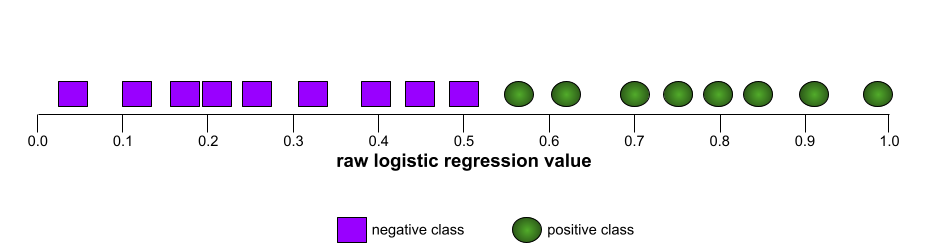The ROC curve for the preceding model looks as follows: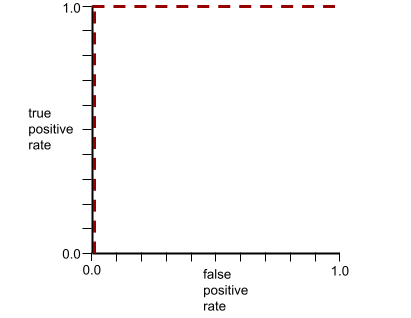In contrast, the following illustration graphs the raw logistic regression values for a terrible model that can't separate negative classes from positive classes at all: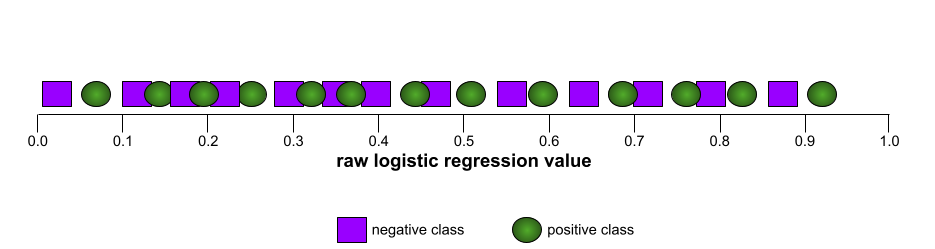The ROC curve for this model looks as follows: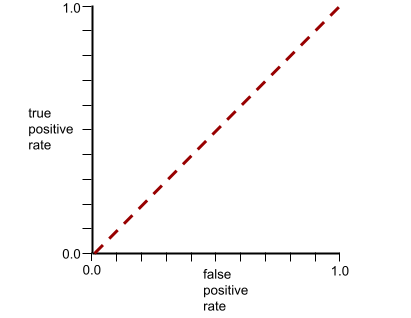Meanwhile, back in the real world, most binary classification models separate positive and negative classes to some degree, but usually not perfectly. So, a typical ROC curve falls somewhere between the two extremes: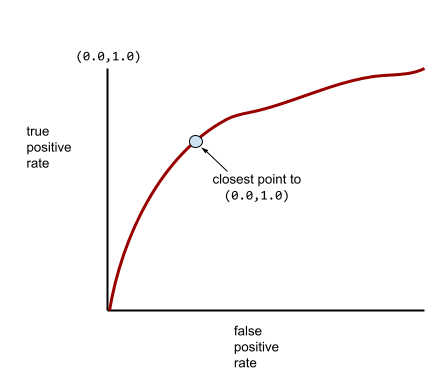The point on an ROC curve closest to (0.0,1.0) theoretically identifies the ideal classification threshold. However, several other real-world issues influence the selection of the ideal classification threshold. For example, perhaps false negatives cause far more pain than false positives.

A numerical metric called AUC summarizes the ROC curve into a single floating-point value.

## role prompting

#language
#generativeAI

An optional part of a prompt that identifies a target audience for a generative AI model's response. Without a role prompt, a large language model provides an answer that may or may not be useful for the person asking the questions. With a role prompt, a large language model can answer in a way that's more appropriate and more helpful for a specific target audience. For example, the role prompt portion of the following prompts are in boldface:

• Describe how tides work for a ten-year old.
• Explain the 2008 financial crisis. Speak as you might to a young child, or a golden retriever.

## root

#df

The starting node (the first condition) in a decision tree. By convention, diagrams put the root at the top of the decision tree. For example: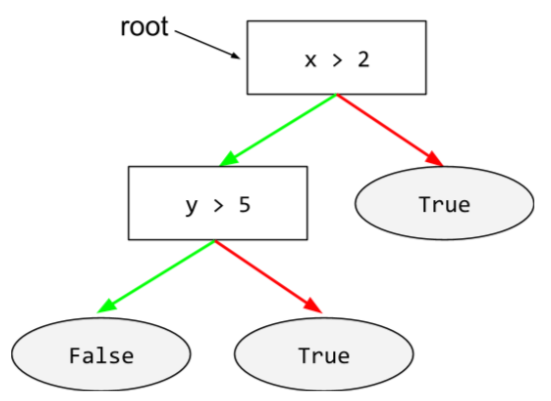## root directory

#TensorFlow

The directory you specify for hosting subdirectories of the TensorFlow checkpoint and events files of multiple models.

## Root Mean Squared Error (RMSE)

#fundamentals

The square root of the Mean Squared Error.

## rotational invariance

#image

In an image classification problem, an algorithm's ability to successfully classify images even when the orientation of the image changes. For example, the algorithm can still identify a tennis racket whether it is pointing up, sideways, or down. Note that rotational invariance is not always desirable; for example, an upside-down 9 should not be classified as a 9.

## R-squared

A regression metric indicating how much variation in a label is due to an individual feature or to a feature set. R-squared is a value between 0 and 1, which you can interpret as follows:

• An R-squared of 0 means that none of a label's variation is due to the feature set.
• An R-squared of 1 means that all of a label's variation is due to the feature set.
• An R-squared between 0 and 1 indicates the extent to which the label's variation can be predicted from a particular feature or the feature set. For example, an R-squared of 0.10 means that 10 percent of the variance in the label is due to the feature set, an R-squared of 0.20 means that 20 percent is due to the feature set, and so on.

R-squared is the square of the Pearson correlation coefficient between the values that a model predicted and ground truth.

## sampling bias

#fairness

See selection bias.

## sampling with replacement

#df

A method of picking items from a set of candidate items in which the same item can be picked multiple times. The phrase "with replacement" means that after each selection, the selected item is returned to the pool of candidate items. The inverse method, sampling without replacement, means that a candidate item can only be picked once.

For example, consider the following fruit set:

fruit = {kiwi, apple, pear, fig, cherry, lime, mango}


Suppose that the system randomly picks fig as the first item. If using sampling with replacement, then the system picks the second item from the following set:

fruit = {kiwi, apple, pear, fig, cherry, lime, mango}


Yes, that's the same set as before, so the system could potentially pick fig again.

If using sampling without replacement, once picked, a sample can't be picked again. For example, if the system randomly picks fig as the first sample, then fig can't be picked again. Therefore, the system picks the second sample from the following (reduced) set:

fruit = {kiwi, apple, pear, cherry, lime, mango}


## SavedModel

#TensorFlow

The recommended format for saving and recovering TensorFlow models. SavedModel is a language-neutral, recoverable serialization format, which enables higher-level systems and tools to produce, consume, and transform TensorFlow models.

See the Saving and Restoring chapter in the TensorFlow Programmer's Guide for complete details.

## Saver

#TensorFlow

A TensorFlow object responsible for saving model checkpoints.

## scalar

A single number or a single string that can be represented as a tensor of rank 0. For example, the following lines of code each create one scalar in TensorFlow:

breed = tf.Variable("poodle", tf.string)
temperature = tf.Variable(27, tf.int16)
precision = tf.Variable(0.982375101275, tf.float64)


## scaling

Any mathematical transform or technique that shifts the range of a label and/or feature value. Some forms of scaling are very useful for transformations like normalization.

Common forms of scaling useful in Machine Learning include:

• linear scaling, which typically uses a combination of subtraction and division to replace the original value with a number between -1 and +1 or between 0 and 1.
• logarithmic scaling, which replaces the original value with its logarithm.
• Z-score normalization, which replaces the original value with a floating-point value representing the number of standard deviations from that feature's mean.

## scikit-learn

A popular open-source machine learning platform. See scikit-learn.org.

## scoring

#recsystems

The part of a recommendation system that provides a value or ranking for each item produced by the candidate generation phase.

## selection bias

#fairness

Errors in conclusions drawn from sampled data due to a selection process that generates systematic differences between samples observed in the data and those not observed. The following forms of selection bias exist:

• coverage bias: The population represented in the dataset doesn't match the population that the machine learning model is making predictions about.
• sampling bias: Data is not collected randomly from the target group.
• non-response bias (also called participation bias): Users from certain groups opt-out of surveys at different rates than users from other groups.

For example, suppose you are creating a machine learning model that predicts people's enjoyment of a movie. To collect training data, you hand out a survey to everyone in the front row of a theater showing the movie. Offhand, this may sound like a reasonable way to gather a dataset; however, this form of data collection may introduce the following forms of selection bias:

• coverage bias: By sampling from a population who chose to see the movie, your model's predictions may not generalize to people who did not already express that level of interest in the movie.
• sampling bias: Rather than randomly sampling from the intended population (all the people at the movie), you sampled only the people in the front row. It is possible that the people sitting in the front row were more interested in the movie than those in other rows.
• non-response bias: In general, people with strong opinions tend to respond to optional surveys more frequently than people with mild opinions. Since the movie survey is optional, the responses are more likely to form a bimodal distribution than a normal (bell-shaped) distribution.

## self-attention (also called self-attention layer)

#language

A neural network layer that transforms a sequence of embeddings (for instance, token embeddings) into another sequence of embeddings. Each embedding in the output sequence is constructed by integrating information from the elements of the input sequence through an attention mechanism.

The self part of self-attention refers to the sequence attending to itself rather than to some other context. Self-attention is one of the main building blocks for Transformers and uses dictionary lookup terminology, such as “query”, “key”, and “value”.

A self-attention layer starts with a sequence of input representations, one for each word. The input representation for a word can be a simple embedding. For each word in an input sequence, the network scores the relevance of the word to every element in the whole sequence of words. The relevance scores determine how much the word's final representation incorporates the representations of other words.

For example, consider the following sentence:

The animal didn't cross the street because it was too tired.

The following illustration (from Transformer: A Novel Neural Network Architecture for Language Understanding) shows a self-attention layer's attention pattern for the pronoun it, with the darkness of each line indicating how much each word contributes to the representation: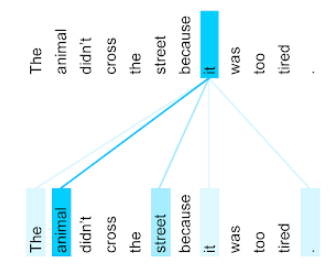The self-attention layer highlights words that are relevant to "it". In this case, the attention layer has learned to highlight words that it might refer to, assigning the highest weight to animal.

For a sequence of n tokens, self-attention transforms a sequence of embeddings n separate times, once at each position in the sequence.

Refer also to attention and multi-head self-attention.

## self-supervised learning

A family of techniques for converting an unsupervised machine learning problem into a supervised machine learning problem by creating surrogate labels from unlabeled examples.

Some Transformer-based models such as BERT use self-supervised learning.

Self-supervised training is a semi-supervised learning approach.

## self-training

A variant of self-supervised learning that is particularly useful when all of the following conditions are true:

Self-training works by iterating over the following two steps until the model stops improving:

1. Use supervised machine learning to train a model on the labeled examples.
2. Use the model created in Step 1 to generate predictions (labels) on the unlabeled examples, moving those in which there is high confidence into the labeled examples with the predicted label.

Notice that each iteration of Step 2 adds more labeled examples for Step 1 to train on.

## semi-supervised learning

Training a model on data where some of the training examples have labels but others don't. One technique for semi-supervised learning is to infer labels for the unlabeled examples, and then to train on the inferred labels to create a new model. Semi-supervised learning can be useful if labels are expensive to obtain but unlabeled examples are plentiful.

Self-training is one technique for semi-supervised learning.

## sensitive attribute

#fairness
A human attribute that may be given special consideration for legal, ethical, social, or personal reasons.

## sentiment analysis

#language

Using statistical or machine learning algorithms to determine a group's overall attitude—positive or negative—toward a service, product, organization, or topic. For example, using natural language understanding, an algorithm could perform sentiment analysis on the textual feedback from a university course to determine the degree to which students generally liked or disliked the course.

## sequence model

#seq

A model whose inputs have a sequential dependence. For example, predicting the next video watched from a sequence of previously watched videos.

#language

A task that converts an input sequence of tokens to an output sequence of tokens. For example, two popular kinds of sequence-to-sequence tasks are:

• Translators:
• Sample input sequence: "I love you."
• Sample output sequence: "Je t'aime."
• Sample input sequence: "Do I need my car in New York City?"

## serving

A synonym for inferring.

## shape (Tensor)

The number of elements in each dimension of a tensor. The shape is represented as a list of integers. For example, the following two-dimensional tensor has a shape of [3,4]:

[[5, 7, 6, 4],
[2, 9, 4, 8],
[3, 6, 5, 1]]


TensorFlow uses row-major (C-style) format to represent the order of dimensions, which is why the shape in TensorFlow is [3,4] rather than [4,3]. In other words, in a two-dimensional TensorFlow Tensor, the shape is [number of rows, number of columns].

## shard

#TensorFlow

A logical division of the training set or the model. Typically, some process creates shards by dividing the examples or parameters into (usually) equal-sized chunks. Each shard is then assigned to a different machine.

Sharding a model is called model parallelism; sharding data is called data parallelism.

## shrinkage

#df

A hyperparameter in gradient boosting that controls overfitting. Shrinkage in gradient boosting is analogous to learning rate in gradient descent. Shrinkage is a decimal value between 0.0 and 1.0. A lower shrinkage value reduces overfitting more than a larger shrinkage value.

## sigmoid function

#fundamentals

A mathematical function that "squishes" an input value into a constrained range, typically 0 to 1 or -1 to +1. That is, you can pass any number (two, a million, negative billion, whatever) to a sigmoid and the output will still be in the constrained range. A plot of the sigmoid activation function looks as follows:The sigmoid function has several uses in machine learning, including:

## similarity measure

#clustering

In clustering algorithms, the metric used to determine how alike (how similar) any two examples are.

## single program / multiple data (SPMD)

A parallelism technique where the same computation is run on different input data in parallel on different devices. The goal of SPMD is to obtain results more quickly. It is the most common style of parallel programming.

## size invariance

#image

In an image classification problem, an algorithm's ability to successfully classify images even when the size of the image changes. For example, the algorithm can still identify a cat whether it consumes 2M pixels or 200K pixels. Note that even the best image classification algorithms still have practical limits on size invariance. For example, an algorithm (or human) is unlikely to correctly classify a cat image consuming only 20 pixels.

## sketching

#clustering

In unsupervised machine learning, a category of algorithms that perform a preliminary similarity analysis on examples. Sketching algorithms use a locality-sensitive hash function to identify points that are likely to be similar, and then group them into buckets.

Sketching decreases the computation required for similarity calculations on large datasets. Instead of calculating similarity for every single pair of examples in the dataset, we calculate similarity only for each pair of points within each bucket.

## softmax

#fundamentals

A function that determines probabilities for each possible class in a multi-class classification model. The probabilities add up to exactly 1.0. For example, the following table shows how softmax distributes various probabilities:

Image is a... Probability
dog .85
cat .13
horse .02

Softmax is also called full softmax.

Contrast with candidate sampling.

## sparse feature

#language
#fundamentals

A feature whose values are predominately zero or empty. For example, a feature containing a single 1 value and a million 0 values is sparse. In contrast, a dense feature has values that are predominantly not zero or empty.

In machine learning, a surprising number of features are sparse features. Categorical features are usually sparse features. For example, of the 300 possible tree species in a forest, a single example might identify just a maple tree. Or, of the millions of possible videos in a video library, a single example might identify just "Casablanca."

In a model, you typically represent sparse features with one-hot encoding. If the one-hot encoding is big, you might put an embedding layer on top of the one-hot encoding for greater efficiency.

## sparse representation

#language
#fundamentals

Storing only the position(s) of nonzero elements in a sparse feature.

For example, suppose a categorical feature named species identifies the 36 tree species in a particular forest. Further assume that each example identifies only a single species.

You could use a one-hot vector to represent the tree species in each example. A one-hot vector would contain a single 1 (to represent the particular tree species in that example) and 35 0s (to represent the 35 tree species not in that example). So, the one-hot representation of maple might look something like the following: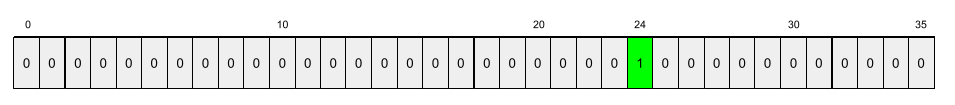Alternatively, sparse representation would simply identify the position of the particular species. If maple is at position 24, then the sparse representation of maple would simply be:

24


Notice that the sparse representation is much more compact than the one-hot representation.

## sparse vector

#fundamentals

A vector whose values are mostly zeroes. See also sparse feature and sparsity.

## sparsity

The number of elements set to zero (or null) in a vector or matrix divided by the total number of entries in that vector or matrix. For example, consider a 100-element matrix in which 98 cells contain zero. The calculation of sparsity is as follows:

$${\text{sparsity}} = \frac{\text{98}} {\text{100}} = {\text{0.98}}$$

Feature sparsity refers to the sparsity of a feature vector; model sparsity refers to the sparsity of the model weights.

#image

See pooling.

## split

#df

In a decision tree, another name for a condition.

## splitter

#df

While training a decision tree, the routine (and algorithm) responsible for finding the best condition at each node.

## SPMD

Abbreviation for single program / multiple data.

## squared hinge loss

The square of the hinge loss. Squared hinge loss penalizes outliers more harshly than regular hinge loss.

## squared loss

#fundamentals

Synonym for L2 loss.

## staged training

#language

A tactic of training a model in a sequence of discrete stages. The goal can be either to speed up the training process, or to achieve better model quality.

An illustration of the progressive stacking approach is shown below:

• Stage 1 contains 3 hidden layers, stage 2 contains 6 hidden layers, and stage 3 contains 12 hidden layers.
• Stage 2 begins training with the weights learned in the 3 hidden layers of Stage 1. Stage 3 begins training with the weights learned in the 6 hidden layers of Stage 2.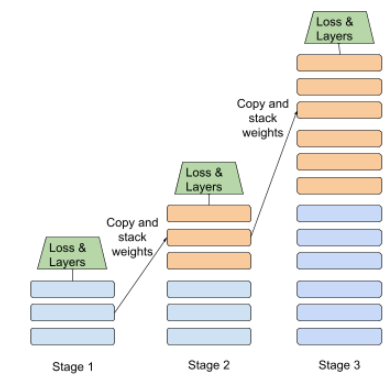## state

#rl

In reinforcement learning, the parameter values that describe the current configuration of the environment, which the agent uses to choose an action.

## state-action value function

#rl

Synonym for Q-function.

## static

#fundamentals

Something done once rather than continuously. The terms static and offline are synonyms. The following are common uses of static and offline in machine learning:

• static model (or offline model) is a model trained once and then used for a while.
• static training (or offline training) is the process of training a static model.
• static inference (or offline inference) is a process in which a model generates a batch of predictions at a time.

Contrast with dynamic.

## static inference

#fundamentals

Synonym for offline inference.

## stationarity

#fundamentals

A feature whose values don't change across one or more dimensions, usually time. For example, a feature whose values look about the same in 2021 and 2023 exhibits stationarity.

In the real world, very few features exhibit stationarity. Even features synonymous with stability (like sea level) change over time.

Contrast with nonstationarity.

## step

A forward pass and backward pass of one batch.

See backpropagation for more information on the forward pass and backward pass.

## step size

Synonym for learning rate.

#fundamentals

A gradient descent algorithm in which the batch size is one. In other words, SGD trains on a single example chosen uniformly at random from a training set.

## stride

#image

In a convolutional operation or pooling, the delta in each dimension of the next series of input slices. For example, the following animation demonstrates a (1,1) stride during a convolutional operation. Therefore, the next input slice starts one position to the right of the previous input slice. When the operation reaches the right edge, the next slice is all the way over to the left but one position down.The preceding example demonstrates a two-dimensional stride. If the input matrix is three-dimensional, the stride would also be three-dimensional.

## structural risk minimization (SRM)

An algorithm that balances two goals:

• The desire to build the most predictive model (for example, lowest loss).
• The desire to keep the model as simple as possible (for example, strong regularization).

For example, a function that minimizes loss+regularization on the training set is a structural risk minimization algorithm.

Contrast with empirical risk minimization.

#image

See pooling.

## summary

#TensorFlow

In TensorFlow, a value or set of values calculated at a particular step, usually used for tracking model metrics during training.

## supervised machine learning

#fundamentals

Training a model from features and their corresponding labels. Supervised machine learning is analogous to learning a subject by studying a set of questions and their corresponding answers. After mastering the mapping between questions and answers, a student can then provide answers to new (never-before-seen) questions on the same topic.

Compare with unsupervised machine learning.

## synthetic feature

#fundamentals

A feature not present among the input features, but assembled from one or more of them. Methods for creating synthetic features include the following:

• Bucketing a continuous feature into range bins.
• Creating a feature cross.
• Multiplying (or dividing) one feature value by other feature value(s) or by itself. For example, if a and b are input features, then the following are examples of synthetic features:
• ab
• a2
• Applying a transcendental function to a feature value. For example, if c is an input feature, then the following are examples of synthetic features:
• sin(c)
• ln(c)

Features created by normalizing or scaling alone are not considered synthetic features.

## T5

#language

A text-to-text transfer learning model introduced by Google AI in 2020. T5 is an encoder-decoder model, based on the Transformer architecture, trained on an extremely large dataset. It is effective at a variety of natural language processing tasks, such as generating text, translating languages, and answering questions in a conversational manner.

T5 gets its name from the five T's in "Text-to-Text Transfer Transformer."

## T5X

#language

An open-source, machine learning framework designed to build and train large-scale natural language processing (NLP) models. T5 is implemented on the T5X codebase (which is built on JAX and Flax).

## tabular Q-learning

#rl

In reinforcement learning, implementing Q-learning by using a table to store the Q-functions for every combination of state and action.

## target

Synonym for label.

## target network

#rl

In Deep Q-learning, a neural network that is a stable approximation of the main neural network, where the main neural network implements either a Q-function or a policy. Then, you can train the main network on the Q-values predicted by the target network. Therefore, you prevent the feedback loop that occurs when the main network trains on Q-values predicted by itself. By avoiding this feedback, training stability increases.

A problem that can be solved using machine learning techniques, such as:

## temperature

#language
#image
#generativeAI

A hyperparameter that controls the degree of randomness of a model's output. Higher temperatures result in more random output, while lower temperatures result in less random output.

Choosing the best temperature depends on the specific application and the desired properties of the model's output. For example, you would probably raise the temperature when creating an application that generates creative output. Conversely, you would probably lower the temperature when building a model that classifies images or text in order to improve the model's accuracy and consistency.

Temperature is often used with softmax.

## temporal data

Data recorded at different points in time. For example, winter coat sales recorded for each day of the year would be temporal data.

## Tensor

#TensorFlow

The primary data structure in TensorFlow programs. Tensors are N-dimensional (where N could be very large) data structures, most commonly scalars, vectors, or matrices. The elements of a Tensor can hold integer, floating-point, or string values.

## TensorBoard

#TensorFlow

The dashboard that displays the summaries saved during the execution of one or more TensorFlow programs.

## TensorFlow

#TensorFlow

A large-scale, distributed, machine learning platform. The term also refers to the base API layer in the TensorFlow stack, which supports general computation on dataflow graphs.

Although TensorFlow is primarily used for machine learning, you may also use TensorFlow for non-ML tasks that require numerical computation using dataflow graphs.

## TensorFlow Playground

#TensorFlow

A program that visualizes how different hyperparameters influence model (primarily neural network) training. Go to http://playground.tensorflow.org to experiment with TensorFlow Playground.

## TensorFlow Serving

#TensorFlow

A platform to deploy trained models in production.

## Tensor Processing Unit (TPU)

#TensorFlow

An application-specific integrated circuit (ASIC) that optimizes the performance of machine learning workloads. These ASICs are deployed as multiple TPU chips on a TPU device.

## Tensor rank

#TensorFlow

See rank (Tensor).

## Tensor shape

#TensorFlow

The number of elements a Tensor contains in various dimensions. For example, a [5, 10] Tensor has a shape of 5 in one dimension and 10 in another.

## Tensor size

#TensorFlow

The total number of scalars a Tensor contains. For example, a [5, 10] Tensor has a size of 50.

## TensorStore

A library for efficiently reading and writing large multi-dimensional arrays.

## termination condition

#rl

In reinforcement learning, the conditions that determine when an episode ends, such as when the agent reaches a certain state or exceeds a threshold number of state transitions. For example, in tic-tac-toe (also known as noughts and crosses), an episode terminates either when a player marks three consecutive spaces or when all spaces are marked.

## test

#df

In a decision tree, another name for a condition.

## test loss

#fundamentals

A metric representing a model's loss against the test set. When building a model, you typically try to minimize test loss. That's because a low test loss is a stronger quality signal than a low training loss or low validation loss.

A large gap between test loss and training loss or validation loss sometimes suggests that you need to increase the regularization rate.

## test set

A subset of the dataset reserved for testing a trained model.

Traditionally, you divide examples in the dataset into the following three distinct subsets:

Each example in a dataset should belong to only one of the preceding subsets. For instance, a single example should not belong to both the training set and the test set.

The training set and validation set are both closely tied to training a model. Because the test set is only indirectly associated with training, test loss is a less biased, higher quality metric than training loss or validation loss.

## text span

#language

The array index span associated with a specific subsection of a text string. For example, the word good in the Python string s="Be good now" occupies the text span from 3 to 6.

## tf.Example

#TensorFlow

A standard protocol buffer for describing input data for machine learning model training or inference.

## tf.keras

#TensorFlow

An implementation of Keras integrated into TensorFlow.

## threshold (for decision trees)

#df

In an axis-aligned condition, the value that a feature is being compared against. For example, 75 is the threshold value in the following condition:

grade >= 75


## time series analysis

#clustering

A subfield of machine learning and statistics that analyzes temporal data. Many types of machine learning problems require time series analysis, including classification, clustering, forecasting, and anomaly detection. For example, you could use time series analysis to forecast the future sales of winter coats by month based on historical sales data.

## timestep

#seq

One "unrolled" cell within a recurrent neural network. For example, the following figure shows three timesteps (labeled with the subscripts t-1, t, and t+1):## token

#language

In a language model, the atomic unit that the model is training on and making predictions on. A token is typically one of the following:

• a word—for example, the phrase "dogs like cats" consists of three word tokens: "dogs", "like", and "cats".
• a character—for example, the phrase "bike fish" consists of nine character tokens. (Note that the blank space counts as one of the tokens.)
• subwords—in which a single word can be a single token or multiple tokens. A subword consists of a root word, a prefix, or a suffix. For example, a language model that uses subwords as tokens might view the word "dogs" as two tokens (the root word "dog" and the plural suffix "s"). That same language model might view the single word "taller" as two subwords (the root word "tall" and the suffix "er").

In domains outside of language models, tokens can represent other kinds of atomic units. For example, in computer vision, a token might be a subset of an image.

## tower

A component of a deep neural network that is itself a deep neural network without an output layer. Typically, each tower reads from an independent data source. Towers are independent until their output is combined in a final layer.

## TPU

#TensorFlow

Abbreviation for Tensor Processing Unit.

## TPU chip

#TensorFlow

A programmable linear algebra accelerator with on-chip high bandwidth memory that is optimized for machine learning workloads. Multiple TPU chips are deployed on a TPU device.

## TPU device

#TensorFlow

A printed circuit board (PCB) with multiple TPU chips, high bandwidth network interfaces, and system cooling hardware.

## TPU master

#TensorFlow

The central coordination process running on a host machine that sends and receives data, results, programs, performance, and system health information to the TPU workers. The TPU master also manages the setup and shutdown of TPU devices.

## TPU node

#TensorFlow

A TPU resource on Google Cloud Platform with a specific TPU type. The TPU node connects to your VPC Network from a peer VPC network. TPU nodes are a resource defined in the Cloud TPU API.

## TPU Pod

#TensorFlow

A specific configuration of TPU devices in a Google data center. All of the devices in a TPU pod are connected to one another over a dedicated high-speed network. A TPU Pod is the largest configuration of TPU devices available for a specific TPU version.

## TPU resource

#TensorFlow

A TPU entity on Google Cloud Platform that you create, manage, or consume. For example, TPU nodes and TPU types are TPU resources.

## TPU slice

#TensorFlow

A TPU slice is a fractional portion of the TPU devices in a TPU Pod. All of the devices in a TPU slice are connected to one another over a dedicated high-speed network.

## TPU type

#TensorFlow

A configuration of one or more TPU devices with a specific TPU hardware version. You select a TPU type when you create a TPU node on Google Cloud Platform. For example, a v2-8 TPU type is a single TPU v2 device with 8 cores. A v3-2048 TPU type has 256 networked TPU v3 devices and a total of 2048 cores. TPU types are a resource defined in the Cloud TPU API.

## TPU worker

#TensorFlow

A process that runs on a host machine and executes machine learning programs on TPU devices.

## training

#fundamentals

The process of determining the ideal parameters (weights and biases) comprising a model. During training, a system reads in examples and gradually adjusts parameters. Training uses each example anywhere from a few times to billions of times.

## training loss

#fundamentals

A metric representing a model's loss during a particular training iteration. For example, suppose the loss function is Mean Squared Error. Perhaps the training loss (the Mean Squared Error) for the 10th iteration is 2.2, and the training loss for the 100th iteration is 1.9.

A loss curve plots training loss vs. the number of iterations. A loss curve provides the following hints about training:

• A downward slope implies that the model is improving.
• An upward slope implies that the model is getting worse.
• A flat slope implies that the model has reached convergence.

For example, the following somewhat idealized loss curve shows:

• A steep downward slope during the initial iterations, which implies rapid model improvement.
• A gradually flattening (but still downward) slope until close to the end of training, which implies continued model improvement at a somewhat slower pace then during the initial iterations.
• A flat slope towards the end of training, which suggests convergence.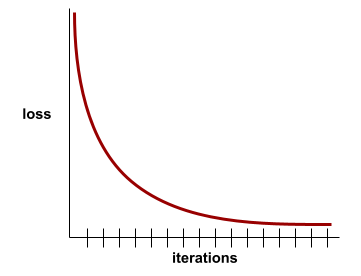## training-serving skew

#fundamentals

The difference between a model's performance during training and that same model's performance during serving.

## training set

#fundamentals

The subset of the dataset used to train a model.

Traditionally, examples in the dataset are divided into the following three distinct subsets:

Ideally, each example in the dataset should belong to only one of the preceding subsets. For example, a single example should not belong to both the training set and the validation set.

## trajectory

#rl

In reinforcement learning, a sequence of tuples that represent a sequence of state transitions of the agent, where each tuple corresponds to the state, action, reward, and next state for a given state transition.

## transfer learning

Transferring information from one machine learning task to another. For example, in multi-task learning, a single model solves multiple tasks, such as a deep model that has different output nodes for different tasks. Transfer learning might involve transferring knowledge from the solution of a simpler task to a more complex one, or involve transferring knowledge from a task where there is more data to one where there is less data.

Most machine learning systems solve a single task. Transfer learning is a baby step towards artificial intelligence in which a single program can solve multiple tasks.

## Transformer

#language

A neural network architecture developed at Google that relies on self-attention mechanisms to transform a sequence of input embeddings into a sequence of output embeddings without relying on convolutions or recurrent neural networks. A Transformer can be viewed as a stack of self-attention layers.

A Transformer can include any of the following:

An encoder transforms a sequence of embeddings into a new sequence of the same length. An encoder includes N identical layers, each of which contains two sub-layers. These two sub-layers are applied at each position of the input embedding sequence, transforming each element of the sequence into a new embedding. The first encoder sub-layer aggregates information from across the input sequence. The second encoder sub-layer transforms the aggregated information into an output embedding.

A decoder transforms a sequence of input embeddings into a sequence of output embeddings, possibly with a different length. A decoder also includes N identical layers with three sub-layers, two of which are similar to the encoder sub-layers. The third decoder sub-layer takes the output of the encoder and applies the self-attention mechanism to gather information from it.

The blog post Transformer: A Novel Neural Network Architecture for Language Understanding provides a good introduction to Transformers.

## translational invariance

#image

In an image classification problem, an algorithm's ability to successfully classify images even when the position of objects within the image changes. For example, the algorithm can still identify a dog, whether it is in the center of the frame or at the left end of the frame.

## trigram

#seq
#language

An N-gram in which N=3.

## true negative (TN)

#fundamentals

An example in which the model correctly predicts the negative class. For example, the model infers that a particular email message is not spam, and that email message really is not spam.

## true positive (TP)

#fundamentals

An example in which the model correctly predicts the positive class. For example, the model infers that a particular email message is spam, and that email message really is spam.

## true positive rate (TPR)

#fundamentals

Synonym for recall. That is:

$$\text{true positive rate} = \frac{\text{true positives}} {\text{true positives} + \text{false negatives}}$$

True positive rate is the y-axis in an ROC curve.

## unawareness (to a sensitive attribute)

#fairness

A situation in which sensitive attributes are present, but not included in the training data. Because sensitive attributes are often correlated with other attributes of one’s data, a model trained with unawareness about a sensitive attribute could still have disparate impact with respect to that attribute, or violate other fairness constraints.

## underfitting

#fundamentals

Producing a model with poor predictive ability because the model hasn't fully captured the complexity of the training data. Many problems can cause underfitting, including:

## undersampling

Removing examples from the majority class in a class-imbalanced dataset in order to create a more balanced training set.

For example, consider a dataset in which the ratio of the majority class to the minority class is 20:1. To overcome this class imbalance, you could create a training set consisting of all of the minority class examples but only a tenth of the majority class examples, which would create a training-set class ratio of 2:1. Thanks to undersampling, this more balanced training set might produce a better model. Alternatively, this more balanced training set might contain insufficient examples to train an effective model.

Contrast with oversampling.

## unidirectional

#language

A system that only evaluates the text that precedes a target section of text. In contrast, a bidirectional system evaluates both the text that precedes and follows a target section of text. See bidirectional for more details.

## unidirectional language model

#language

A language model that bases its probabilities only on the tokens appearing before, not after, the target token(s). Contrast with bidirectional language model.

## unlabeled example

#fundamentals

An example that contains features but no label. For example, the following table shows three unlabeled examples from a house valuation model, each with three features but no house value:

Number of bedrooms Number of bathrooms House age
3 2 15
2 1 72
4 2 34

In supervised machine learning, models train on labeled examples and make predictions on unlabeled examples.

In semi-supervised and unsupervised learning, unlabeled examples are used during training.

Contrast unlabeled example with labeled example.

## unsupervised machine learning

#clustering
#fundamentals

Training a model to find patterns in a dataset, typically an unlabeled dataset.

The most common use of unsupervised machine learning is to cluster data into groups of similar examples. For example, an unsupervised machine learning algorithm can cluster songs based on various properties of the music. The resulting clusters can become an input to other machine learning algorithms (for example, to a music recommendation service). Clustering can help when useful labels are scarce or absent. For example, in domains such as anti-abuse and fraud, clusters can help humans better understand the data.

Contrast with supervised machine learning.

## uplift modeling

A modeling technique, commonly used in marketing, that models the "causal effect" (also known as the "incremental impact") of a "treatment" on an "individual." Here are two examples:

• Doctors might use uplift modeling to predict the mortality decrease (causal effect) of a medical procedure (treatment) depending on the age and medical history of a patient (individual).
• Marketers might use uplift modeling to predict the increase in probability of a purchase (causal effect) due to an advertisement (treatment) on a person (individual).

Uplift modeling differs from classification or regression in that some labels (for example, half of the labels in binary treatments) are always missing in uplift modeling. For example, a patient can either receive or not receive a treatment; therefore, we can only observe whether the patient is going to heal or not heal in only one of these two situations (but never both). The main advantage of an uplift model is that it can generate predictions for the unobserved situation (the counterfactual) and use it to compute the causal effect.

## upweighting

Applying a weight to the downsampled class equal to the factor by which you downsampled.

## user matrix

#recsystems

In recommendation systems, an embedding vector generated by matrix factorization that holds latent signals about user preferences. Each row of the user matrix holds information about the relative strength of various latent signals for a single user. For example, consider a movie recommendation system. In this system, the latent signals in the user matrix might represent each user's interest in particular genres, or might be harder-to-interpret signals that involve complex interactions across multiple factors.

The user matrix has a column for each latent feature and a row for each user. That is, the user matrix has the same number of rows as the target matrix that is being factorized. For example, given a movie recommendation system for 1,000,000 users, the user matrix will have 1,000,000 rows.

## validation

#fundamentals

The initial evaluation of a model's quality. Validation checks the quality of a model's predictions against the validation set.

Because the validation set differs from the training set, validation helps guard against overfitting.

You might think of evaluating the model against the validation set as the first round of testing and evaluating the model against the test set as the second round of testing.

## validation loss

#fundamentals

A metric representing a model's loss on the validation set during a particular iteration of training.

## validation set

#fundamentals

The subset of the dataset that performs initial evaluation against a trained model. Typically, you evaluate the trained model against the validation set several times before evaluating the model against the test set.

Traditionally, you divide the examples in the dataset into the following three distinct subsets:

Ideally, each example in the dataset should belong to only one of the preceding subsets. For example, a single example should not belong to both the training set and the validation set.

## value imputation

The process of replacing a missing value with an acceptable substitute. When a value is missing, you can either discard the entire example or you can use value imputation to salvage the example.

For example, consider a dataset containing a temperature feature that is supposed to be recorded every hour. However, the temperature reading was unavailable for a particular hour. Here is a section of the dataset:

Timestamp Temperature
1680561000 10
1680564600 12
1680568200 missing
1680571800 20
1680575400 21
1680579000 21

A system could either delete the missing example or impute the missing temperature as 12, 16, 18, or 20, depending on the imputation algorithm.

#seq

The tendency for the gradients of early hidden layers of some deep neural networks to become surprisingly flat (low). Increasingly lower gradients result in increasingly smaller changes to the weights on nodes in a deep neural network, leading to little or no learning. Models suffering from the vanishing gradient problem become difficult or impossible to train. Long Short-Term Memory cells address this issue.

## variable importances

#df

A set of scores that indicates the relative importance of each feature to the model.

For example, consider a decision tree that estimates house prices. Suppose this decision tree uses three features: size, age, and style. If a set of variable importances for the three features are calculated to be {size=5.8, age=2.5, style=4.7}, then size is more important to the decision tree than age or style.

Different variable importance metrics exist, which can inform ML experts about different aspects of models.

## variational autoencoder (VAE)

#language

A type of autoencoder that leverages the discrepancy between inputs and outputs to generate modified versions of the inputs. Variational autoencoders are useful for generative AI.

VAEs are based on variational inference: a technique for estimating the parameters of a probability model.

## Wasserstein loss

One of the loss functions commonly used in generative adversarial networks, based on the earth mover's distance between the distribution of generated data and real data.

## weight

#fundamentals

A value that a model multiplies by another value. Training is the process of determining a model's ideal weights; inference is the process of using those learned weights to make predictions.

## Weighted Alternating Least Squares (WALS)

#recsystems

An algorithm for minimizing the objective function during matrix factorization in recommendation systems, which allows a downweighting of the missing examples. WALS minimizes the weighted squared error between the original matrix and the reconstruction by alternating between fixing the row factorization and column factorization. Each of these optimizations can be solved by least squares convex optimization. For details, see the Recommendation Systems course.

## weighted sum

#fundamentals

The sum of all the relevant input values multiplied by their corresponding weights. For example, suppose the relevant inputs consist of the following:

 input value input weight 2 -1.3 -1 0.6 3 0.4

The weighted sum is therefore:

weighted sum = (2)(-1.3) + (-1)(0.6) + (3)(0.4) = -2.0


A weighted sum is the input argument to an activation function.

## wide model

A linear model that typically has many sparse input features. We refer to it as "wide" since such a model is a special type of neural network with a large number of inputs that connect directly to the output node. Wide models are often easier to debug and inspect than deep models. Although wide models cannot express nonlinearities through hidden layers, wide models can use transformations such as feature crossing and bucketization to model nonlinearities in different ways.

Contrast with deep model.

## width

The number of neurons in a particular layer of a neural network.

## wisdom of the crowd

#df

The idea that averaging the opinions or estimates of a large group of people ("the crowd") often produces surprisingly good results. For example, consider a game in which people guess the number of jelly beans packed into a large jar. Although most individual guesses will be inaccurate, the average of all the guesses has been empirically shown to be surprisingly close to the actual number of jelly beans in the jar.

Ensembles are a software analog of wisdom of the crowd. Even if individual models make wildly inaccurate predictions, averaging the predictions of many models often generates surprisingly good predictions. For example, although an individual decision tree might make poor predictions, a decision forest often makes very good predictions.

## word embedding

#language

Representing each word in a word set within an embedding vector; that is, representing each word as a vector of floating-point values between 0.0 and 1.0. Words with similar meanings have more-similar representations than words with different meanings. For example, carrots, celery, and cucumbers would all have relatively similar representations, which would be very different from the representations of airplane, sunglasses, and toothpaste.

## XLA (Accelerated Linear Algebra)

An open-source machine learning compiler for GPUs, CPUs, and ML accelerators.

The XLA compiler takes models from popular ML frameworks such as PyTorch, TensorFlow, and JAX, and optimizes them for high-performance execution across different hardware platforms including GPUs, CPUs, and ML accelerators.

## zero-shot learning

A type of machine learning training where the model infers a prediction for a task that it was not specifically already trained on. In other words, the model is given zero task-specific training examples but asked to do inference for that task.

## zero-shot prompting

#language
#generativeAI

A prompt that does not provide an example of how you want the large language model to respond. For example:

Parts of one prompt Notes
What is the official currency of the specified country? The question you want the LLM to answer.
India: The actual query.

The large language model might respond with any of the following:

• Rupee
• INR
• Indian rupee
• The rupee
• The Indian rupee

All of the answers are correct, though you might prefer a particular format.

Compare and contrast zero-shot prompting with the following terms:

## Z-score normalization

#fundamentals

A scaling technique that replaces a raw feature value with a floating-point value representing the number of standard deviations from that feature's mean. For example, consider a feature whose mean is 800 and whose standard deviation is 100. The following table shows how Z-score normalization would map the raw value to its Z-score:

Raw value Z-score
800 0
950 +1.5
575 -2.25

The machine learning model then trains on the Z-scores for that feature instead of on the raw values.

[{ "type": "thumb-down", "id": "missingTheInformationINeed", "label":"Missing the information I need" },{ "type": "thumb-down", "id": "tooComplicatedTooManySteps", "label":"Too complicated / too many steps" },{ "type": "thumb-down", "id": "outOfDate", "label":"Out of date" },{ "type": "thumb-down", "id": "samplesCodeIssue", "label":"Samples / code issue" },{ "type": "thumb-down", "id": "otherDown", "label":"Other" }]
[{ "type": "thumb-up", "id": "easyToUnderstand", "label":"Easy to understand" },{ "type": "thumb-up", "id": "solvedMyProblem", "label":"Solved my problem" },{ "type": "thumb-up", "id": "otherUp", "label":"Other" }]